# Concise Selina Solutions for Class 9 Maths Chapter 5- Factorisation

Selina Solutions are considered to be very useful when you are preparing for the ICSE Class 9 Maths exams. Here, we bring to you detailed answers and solutions to the exercises of Selina Solutions for Class 9 Maths Chapter 5- Factorisation. These questions have been devised by the subject matter experts, as per the syllabus prescribed by the CISCE for the ICSE.

Here, the PDF of the Class 9 Maths Chapter 5 Selina solutions is available which can be downloaded as well as viewed online. Students can also avail these Selina solutions and download it for free to practise them offline as well.

## Download PDF of Selina Solutions for Class 9 Maths Chapter 5:-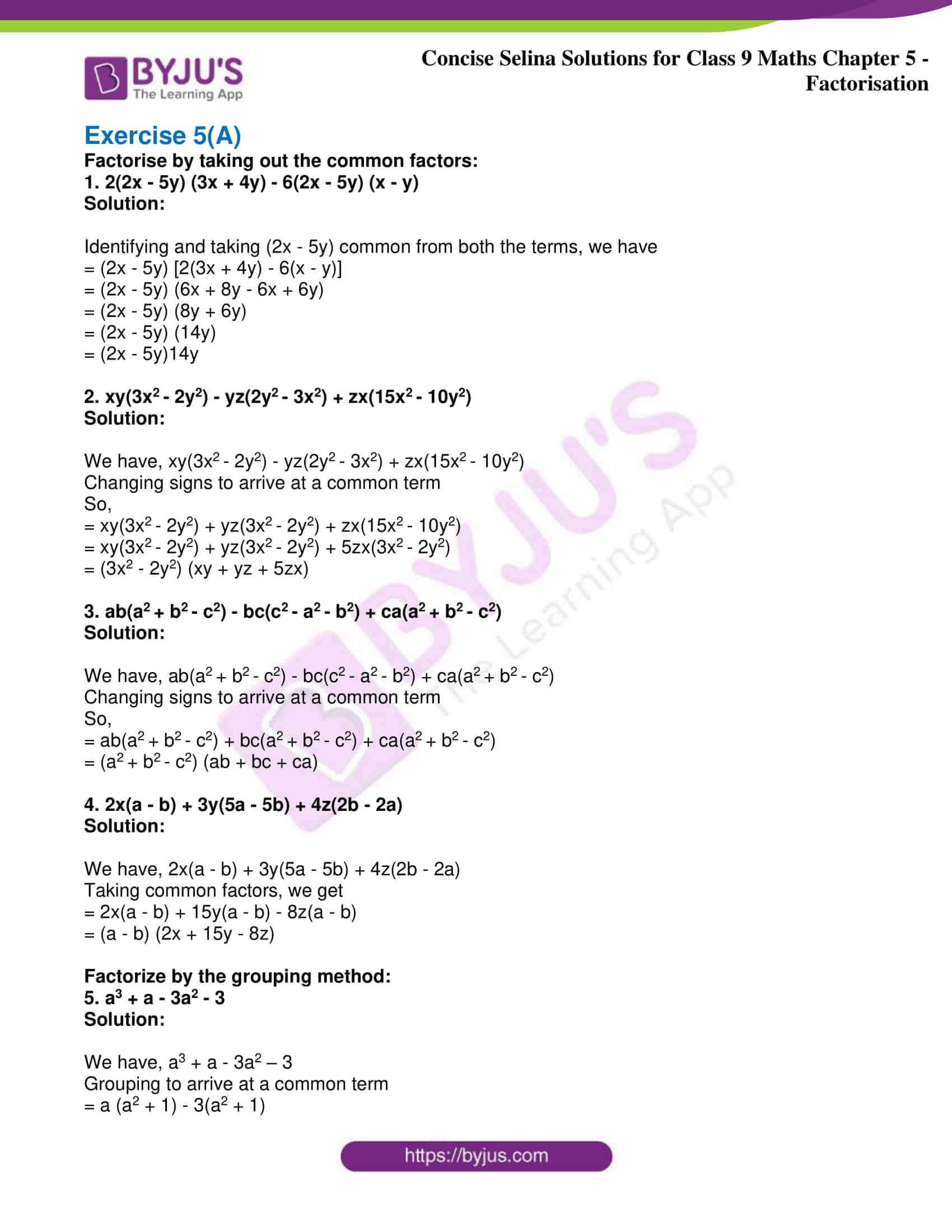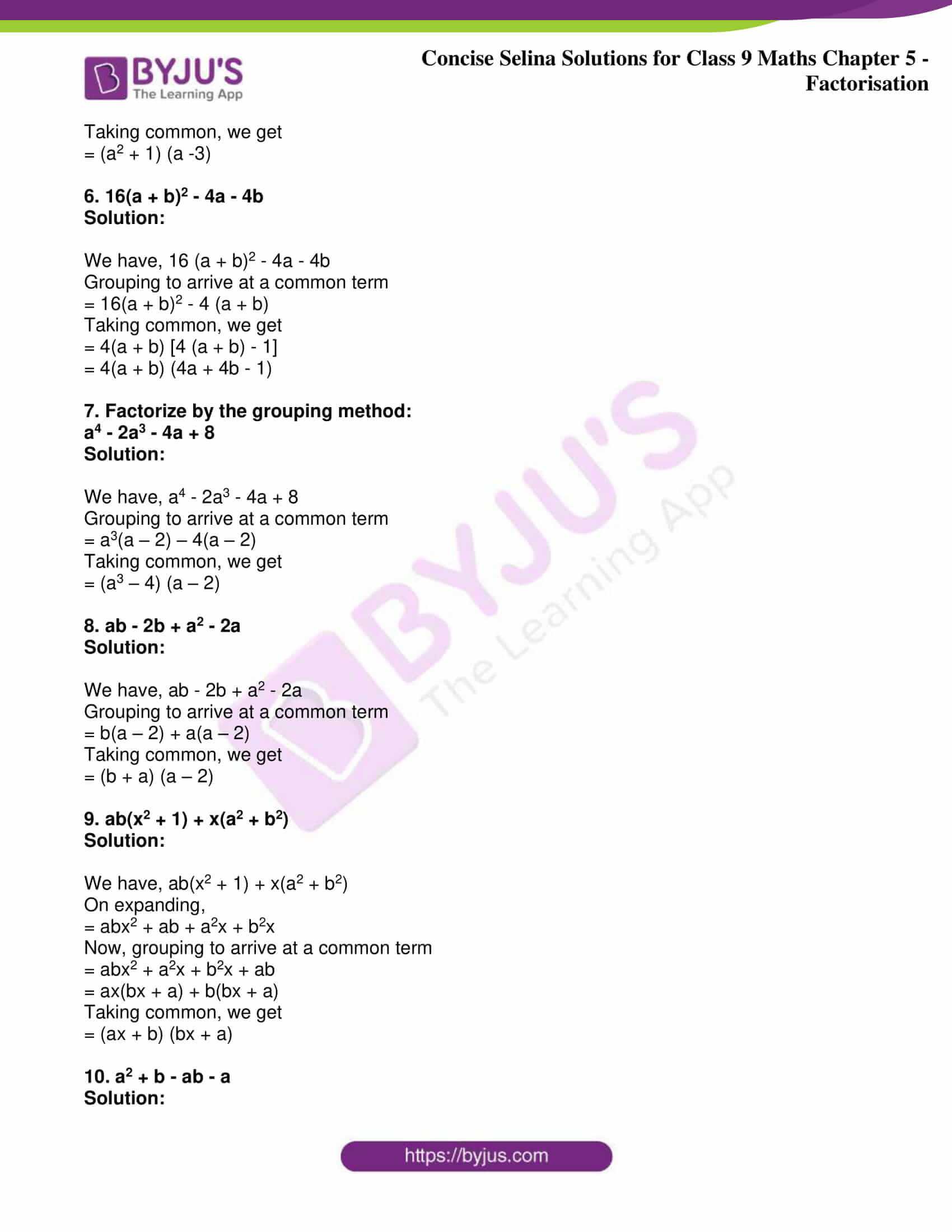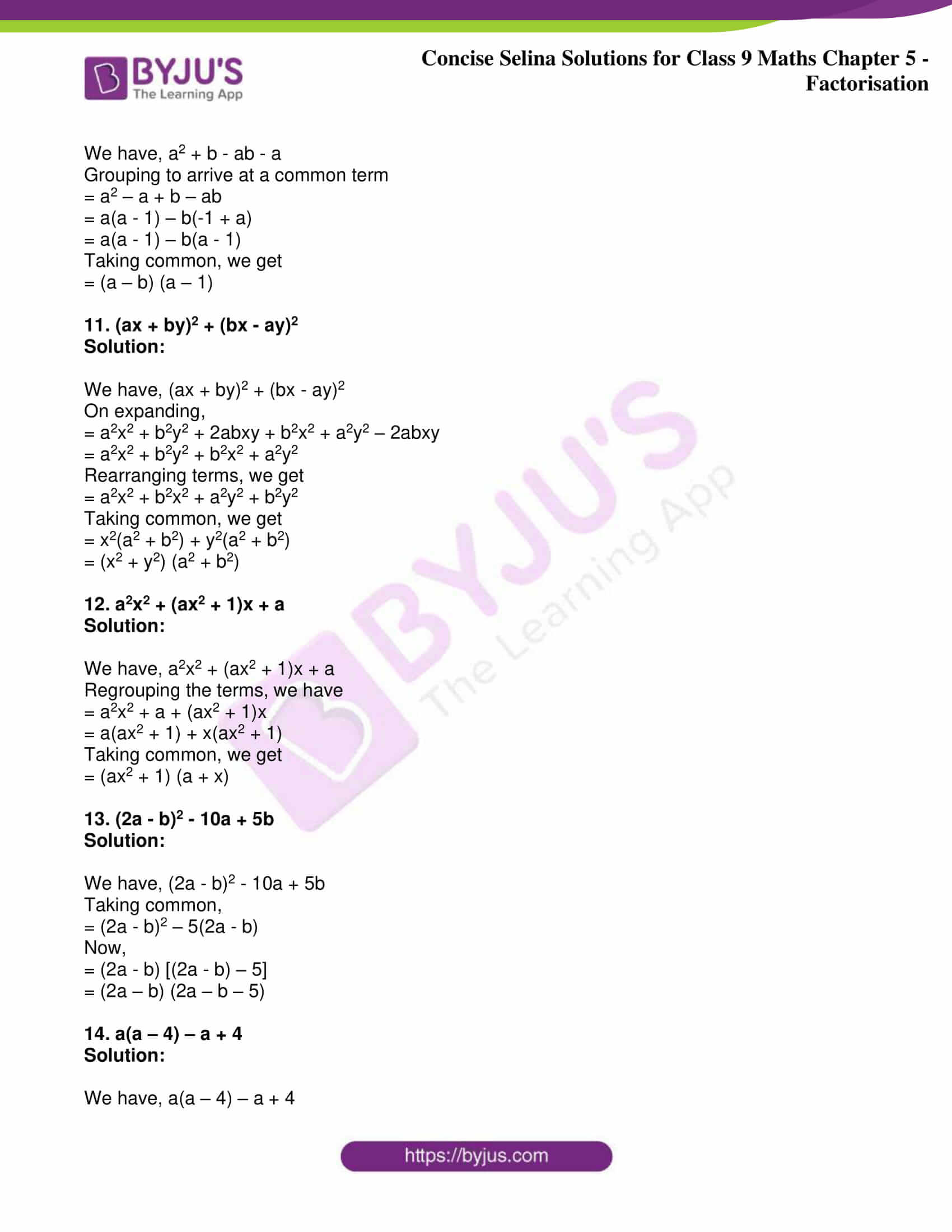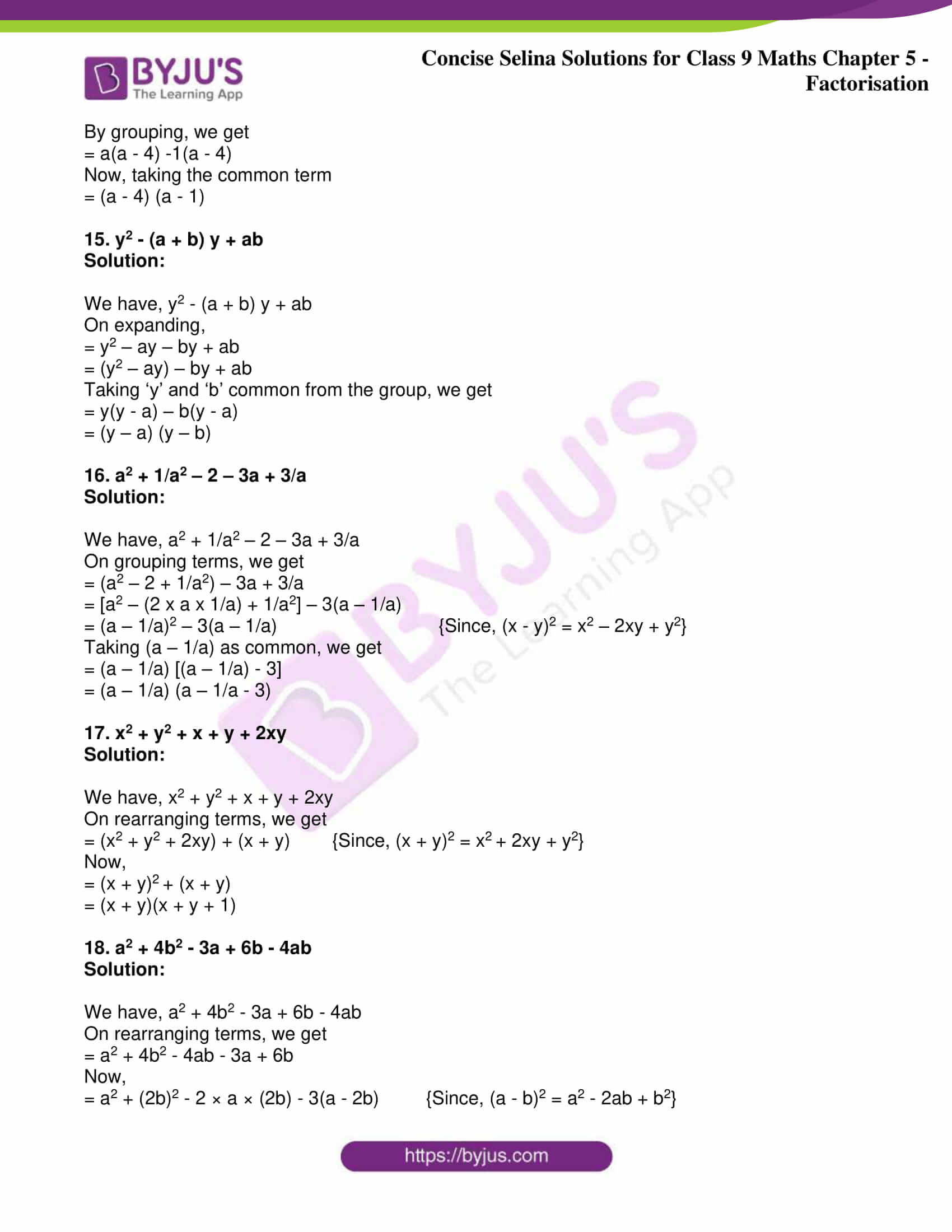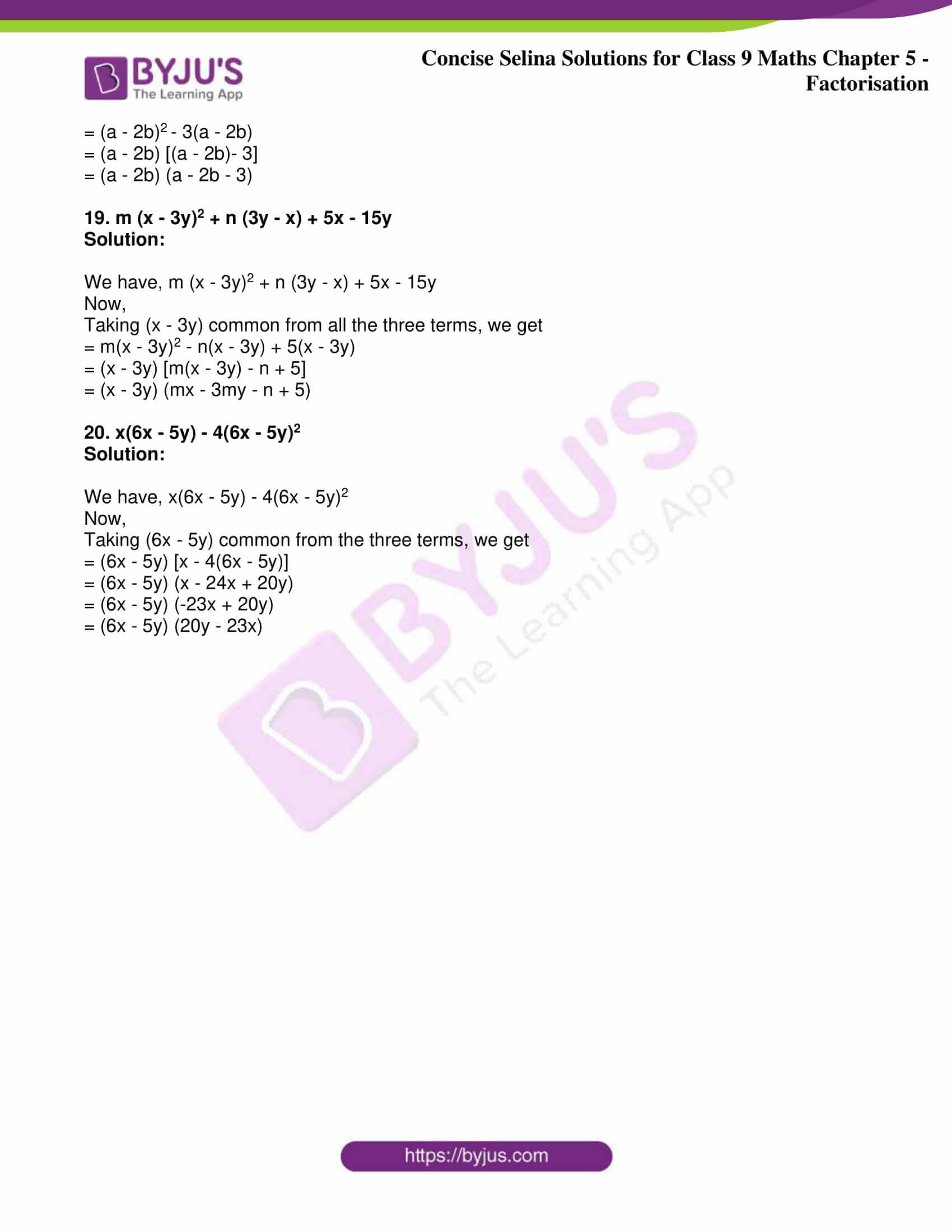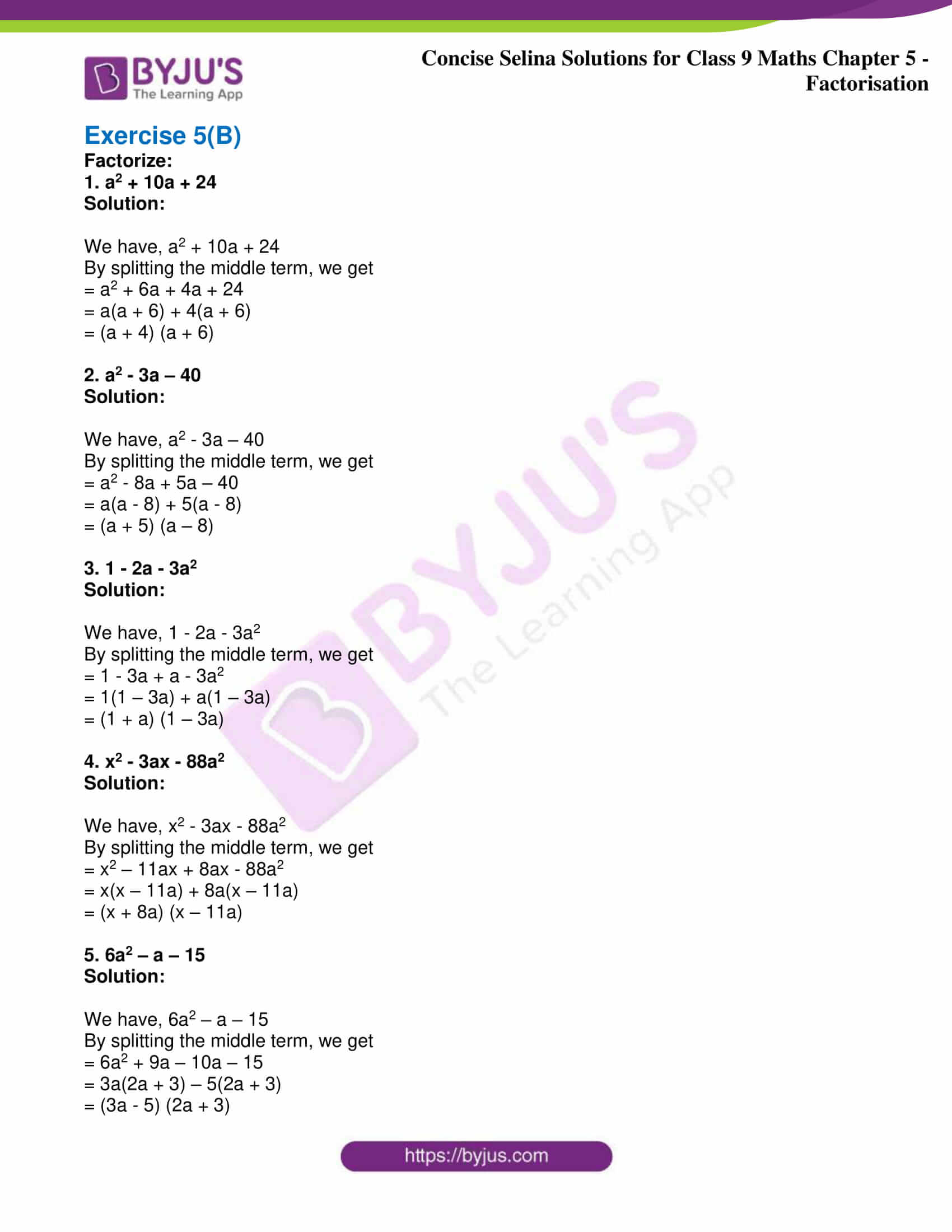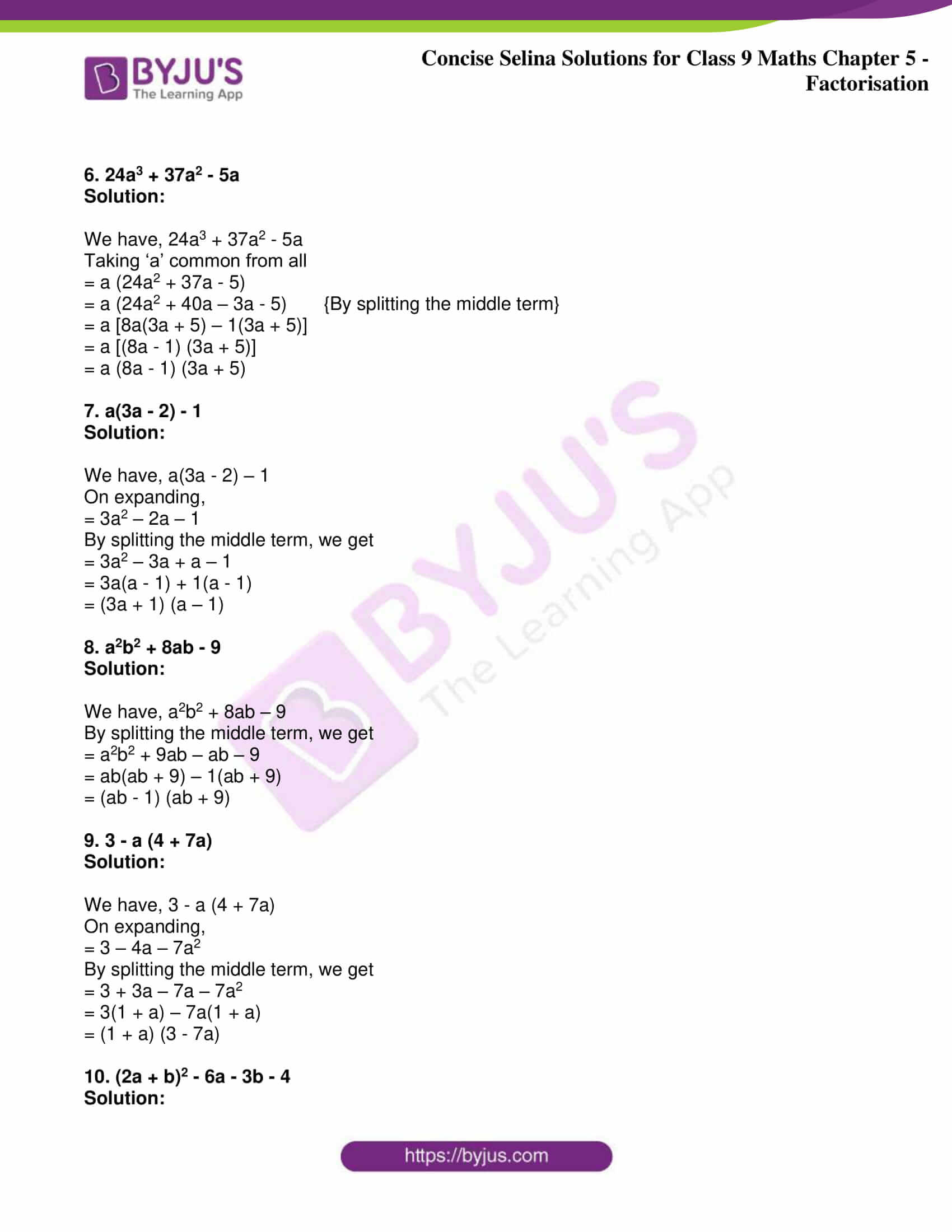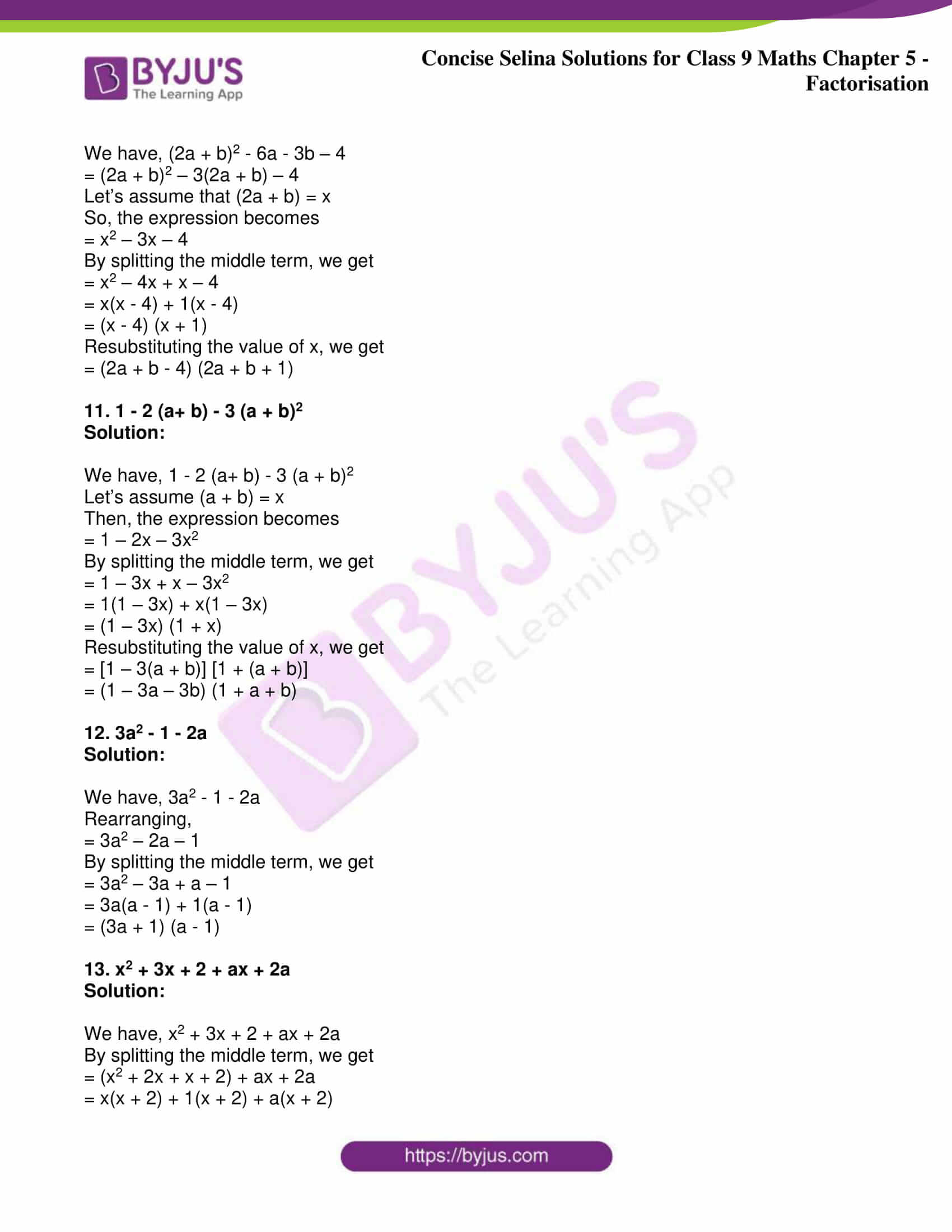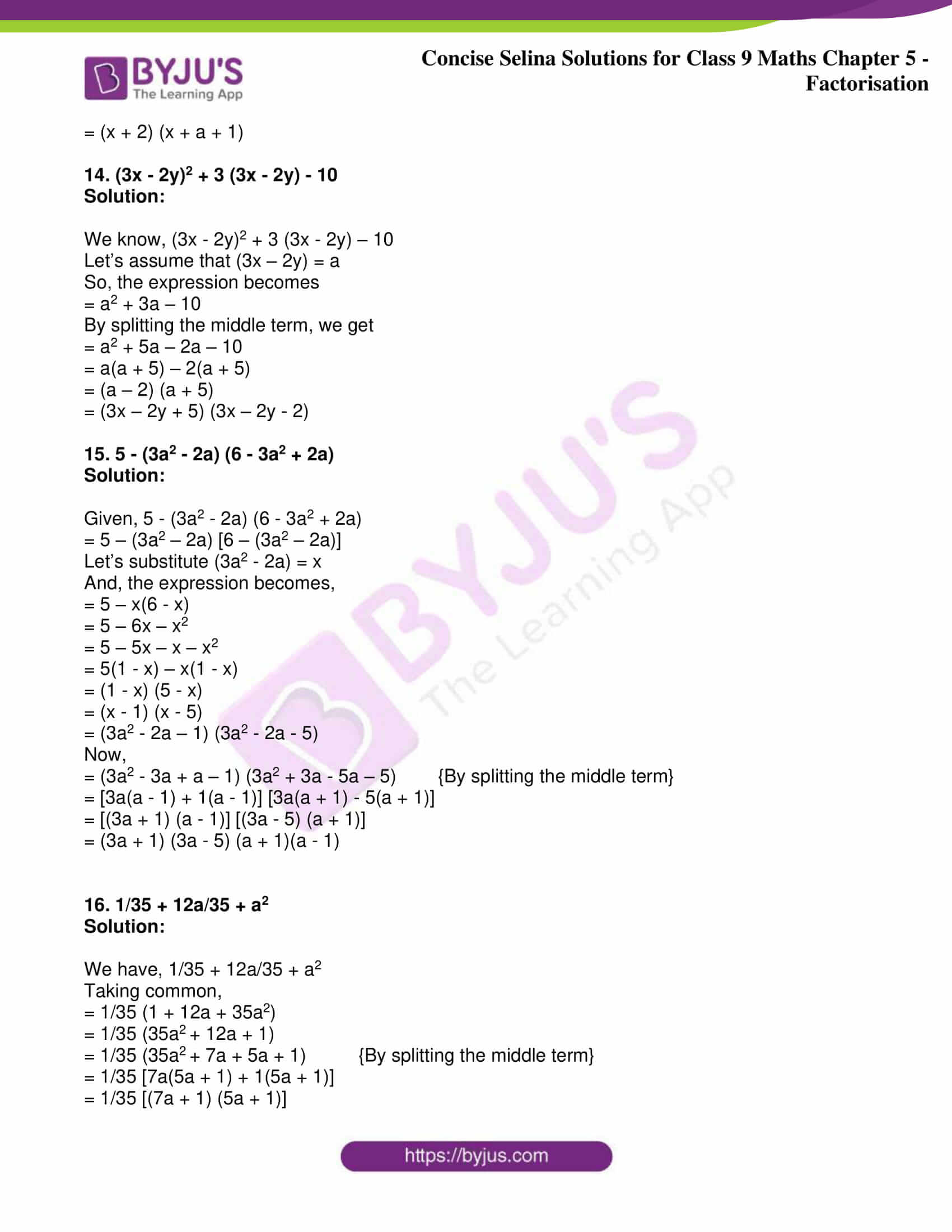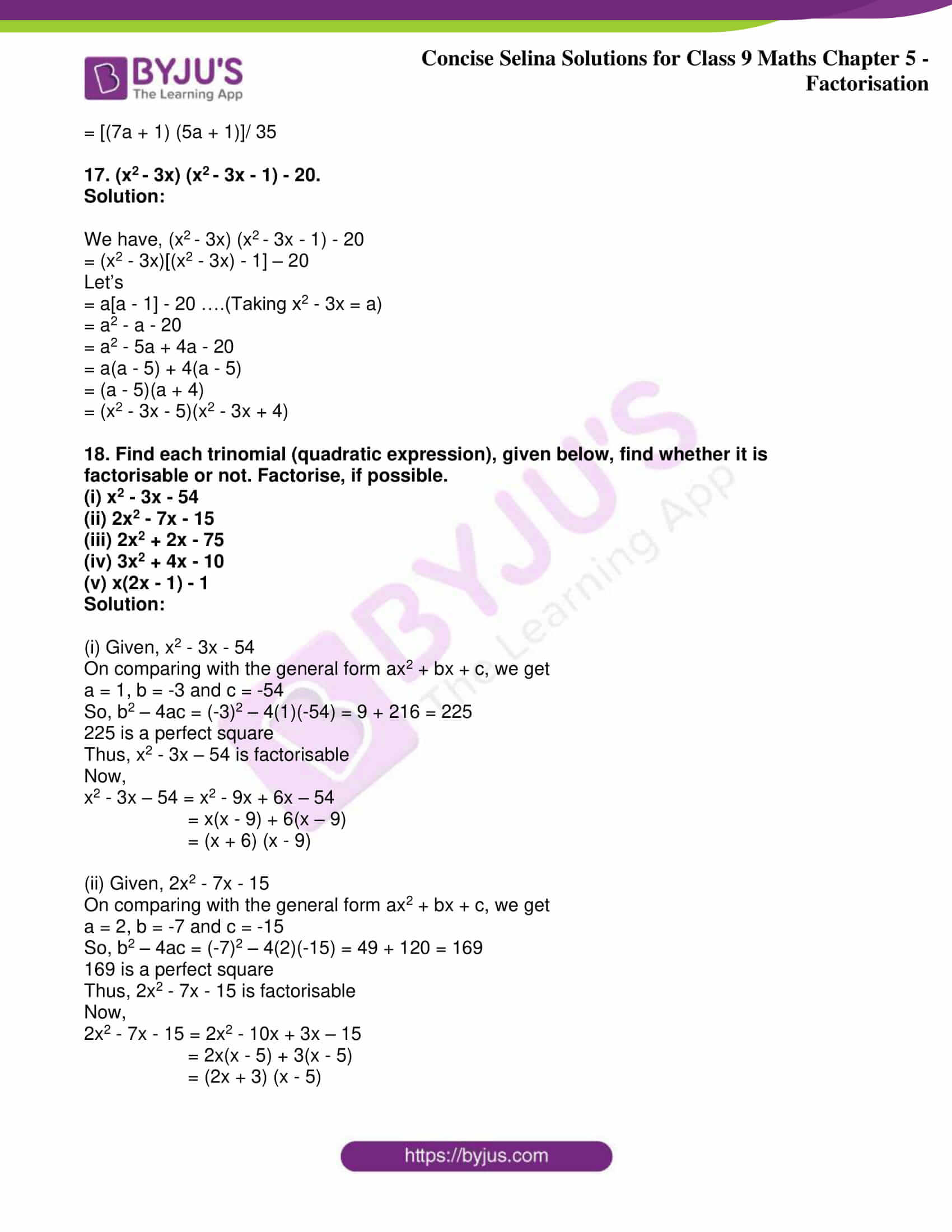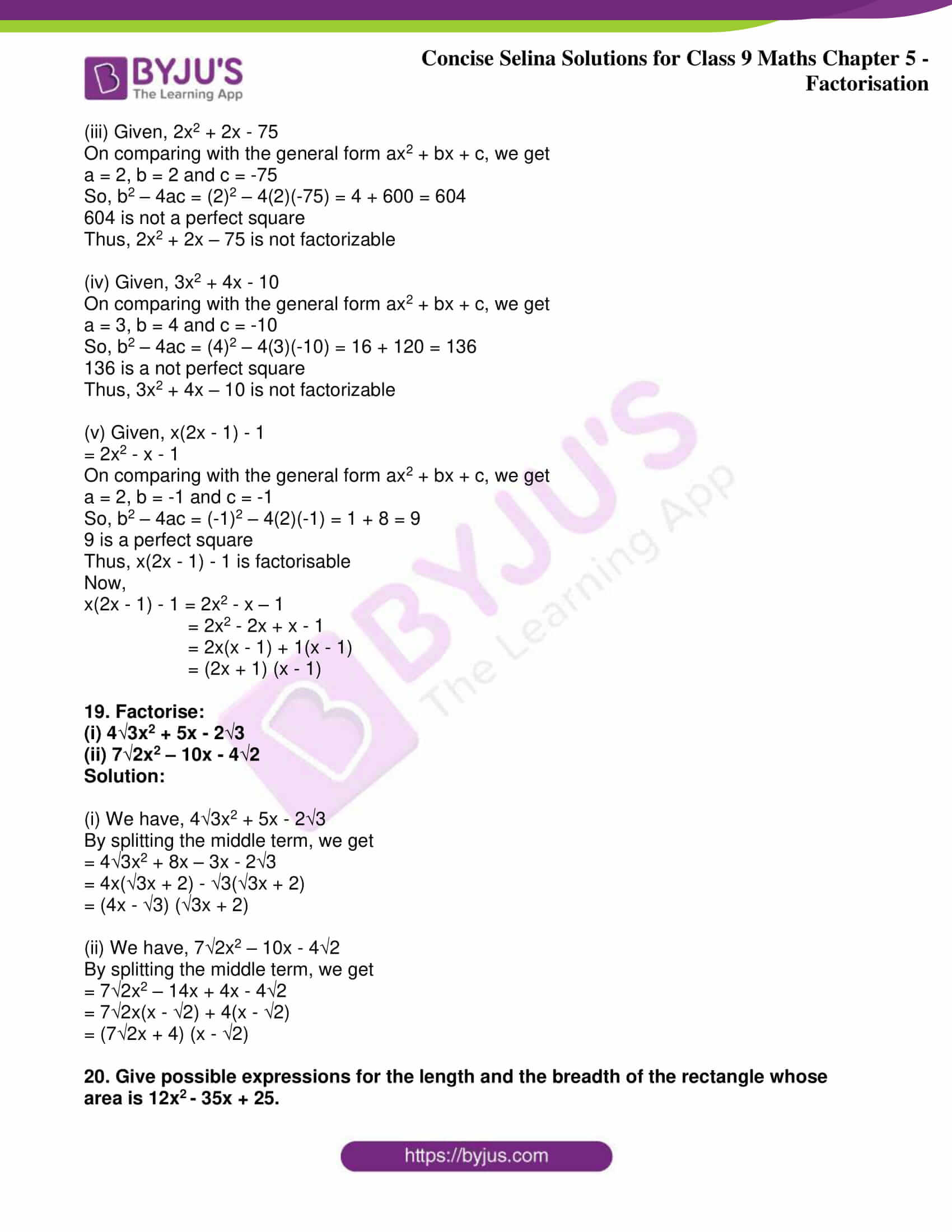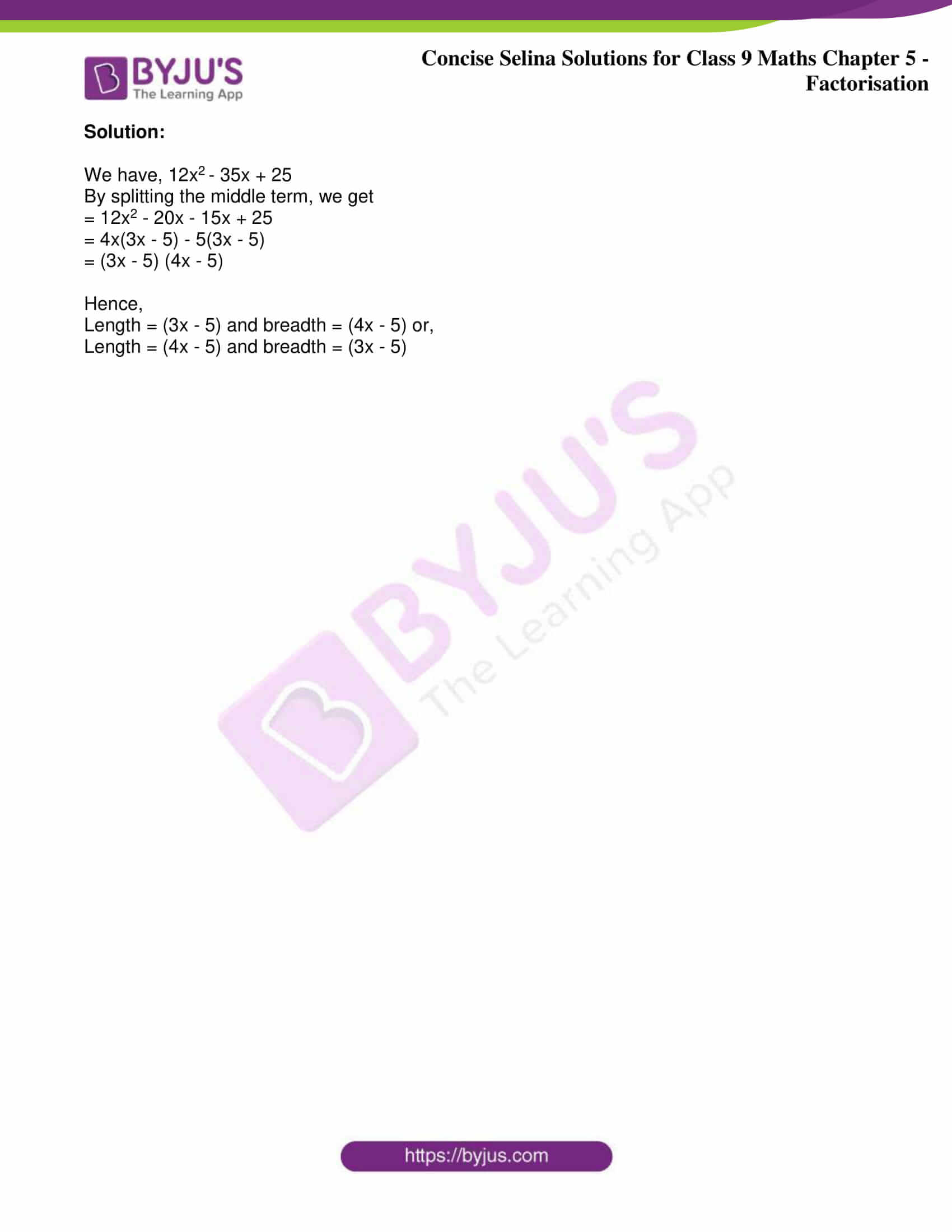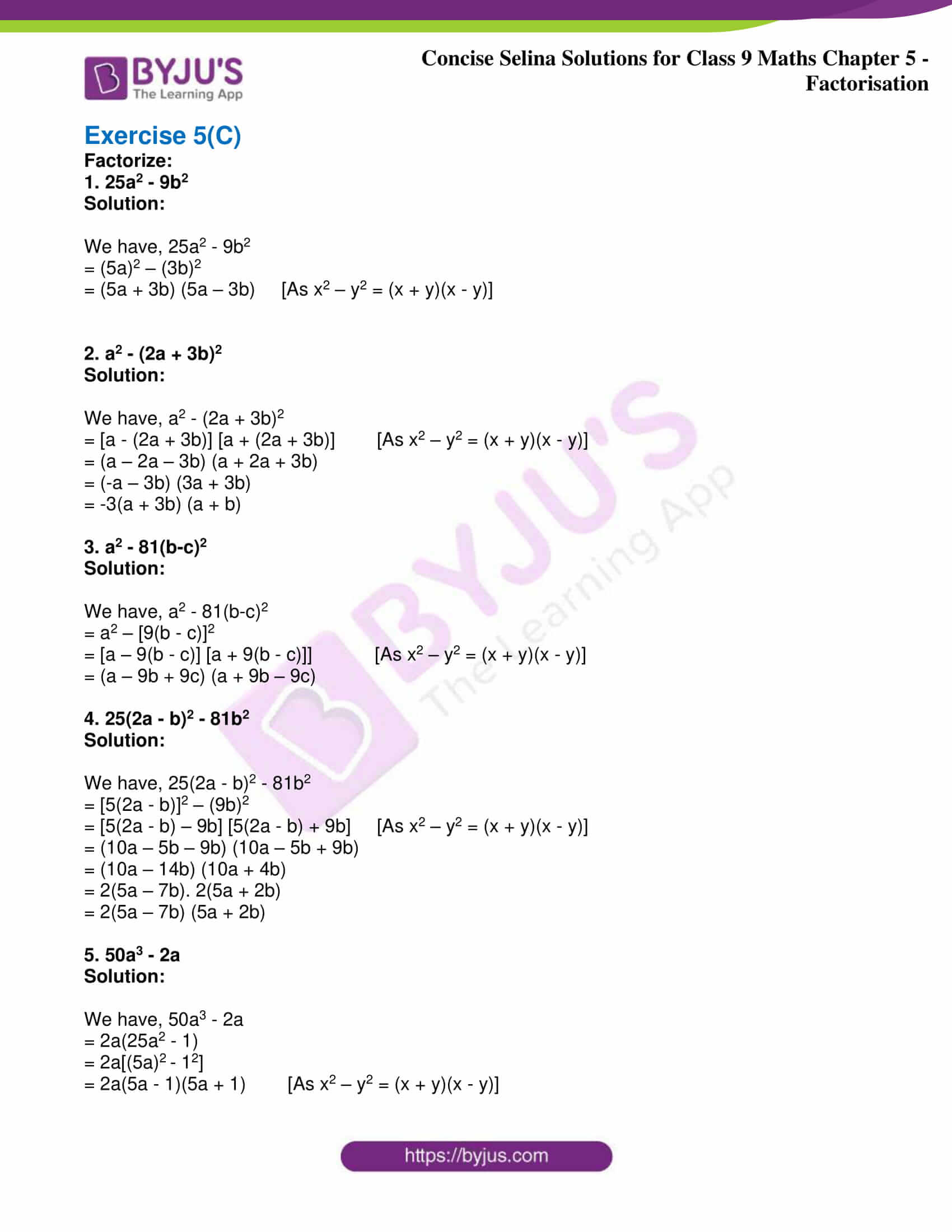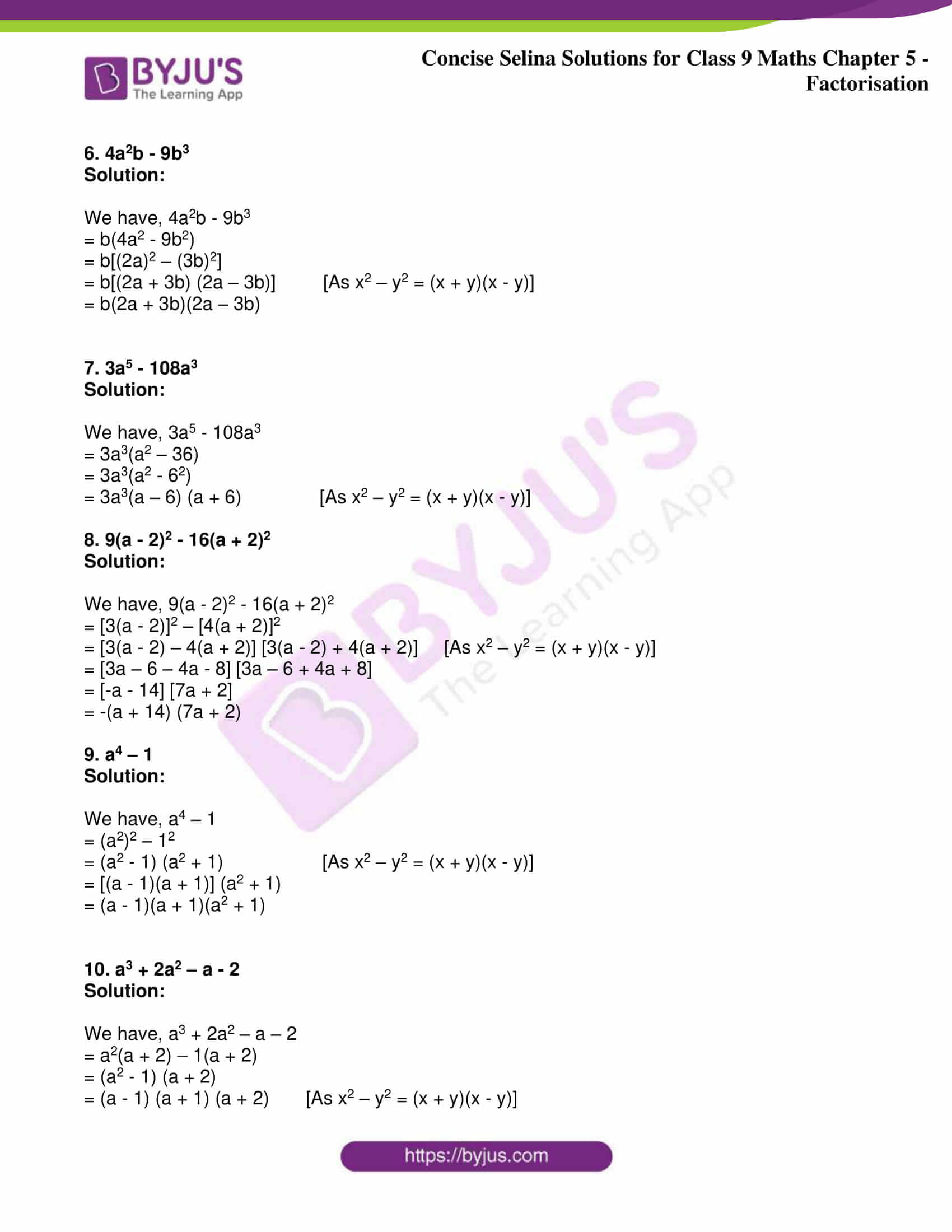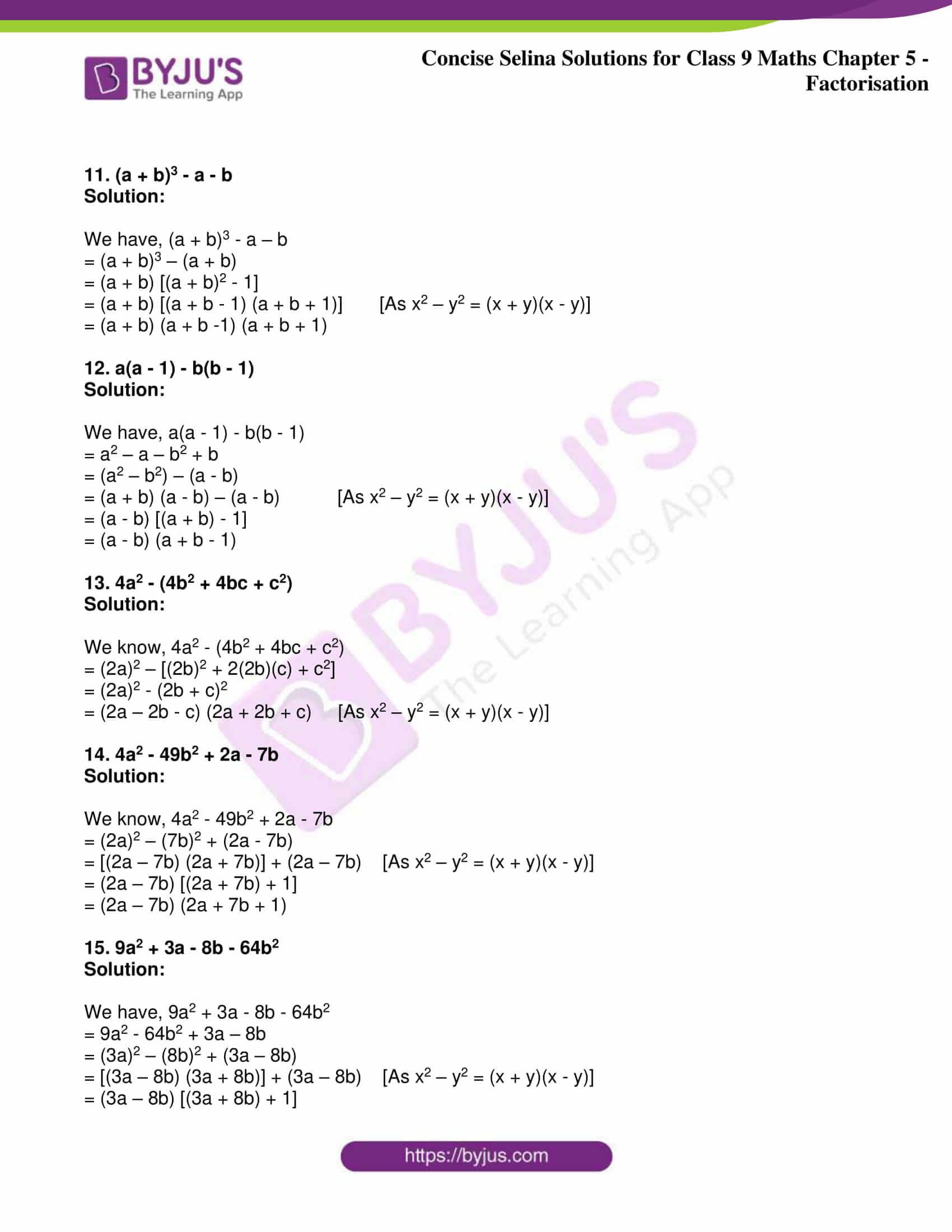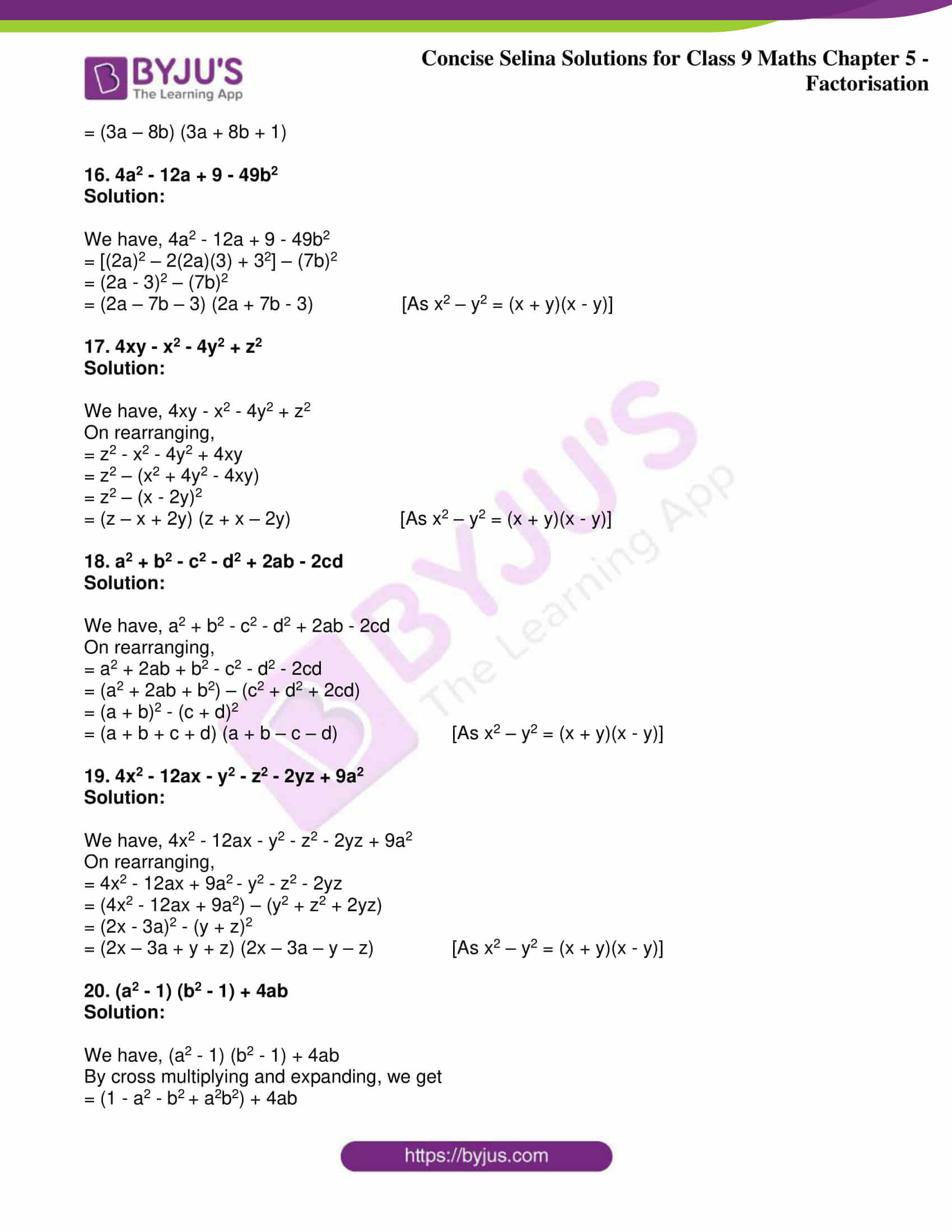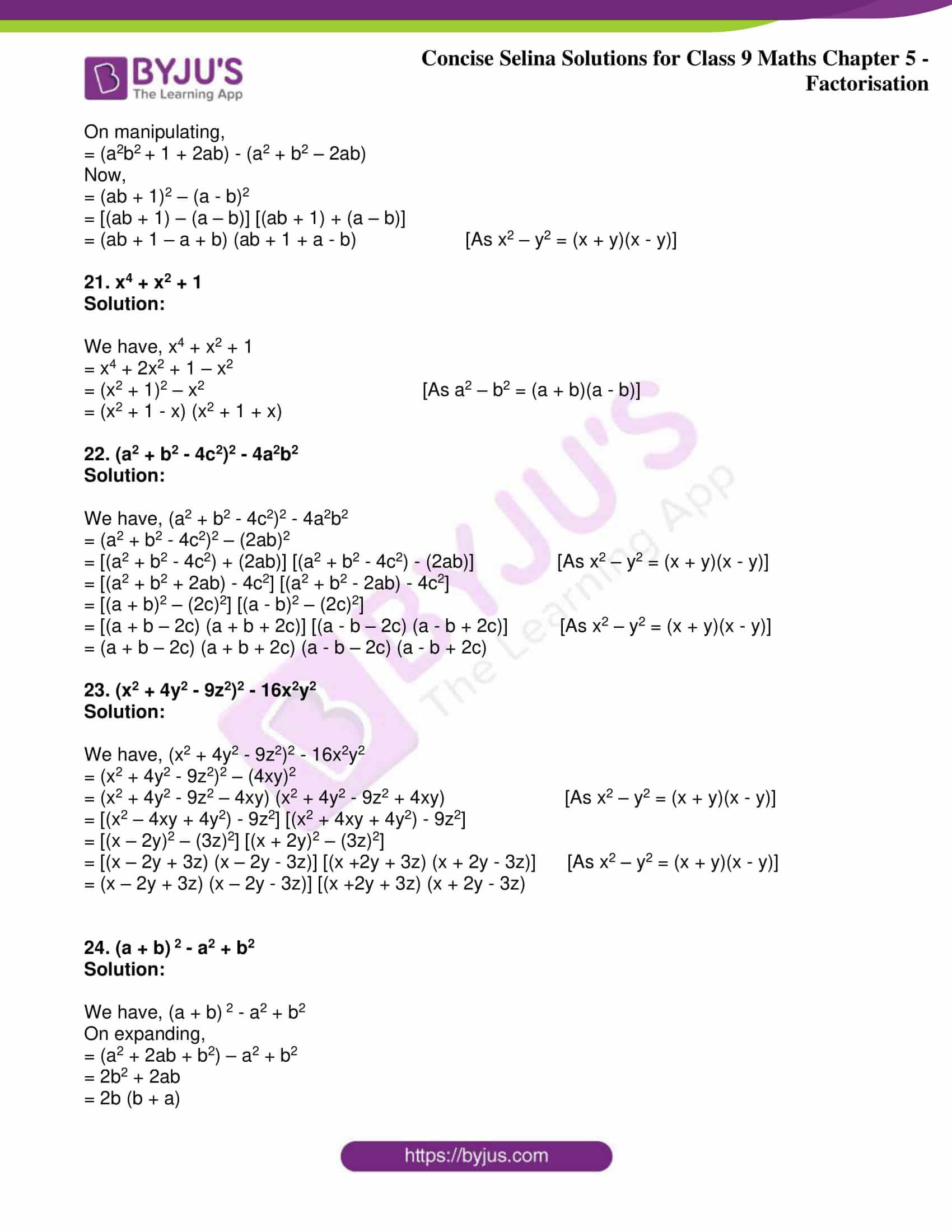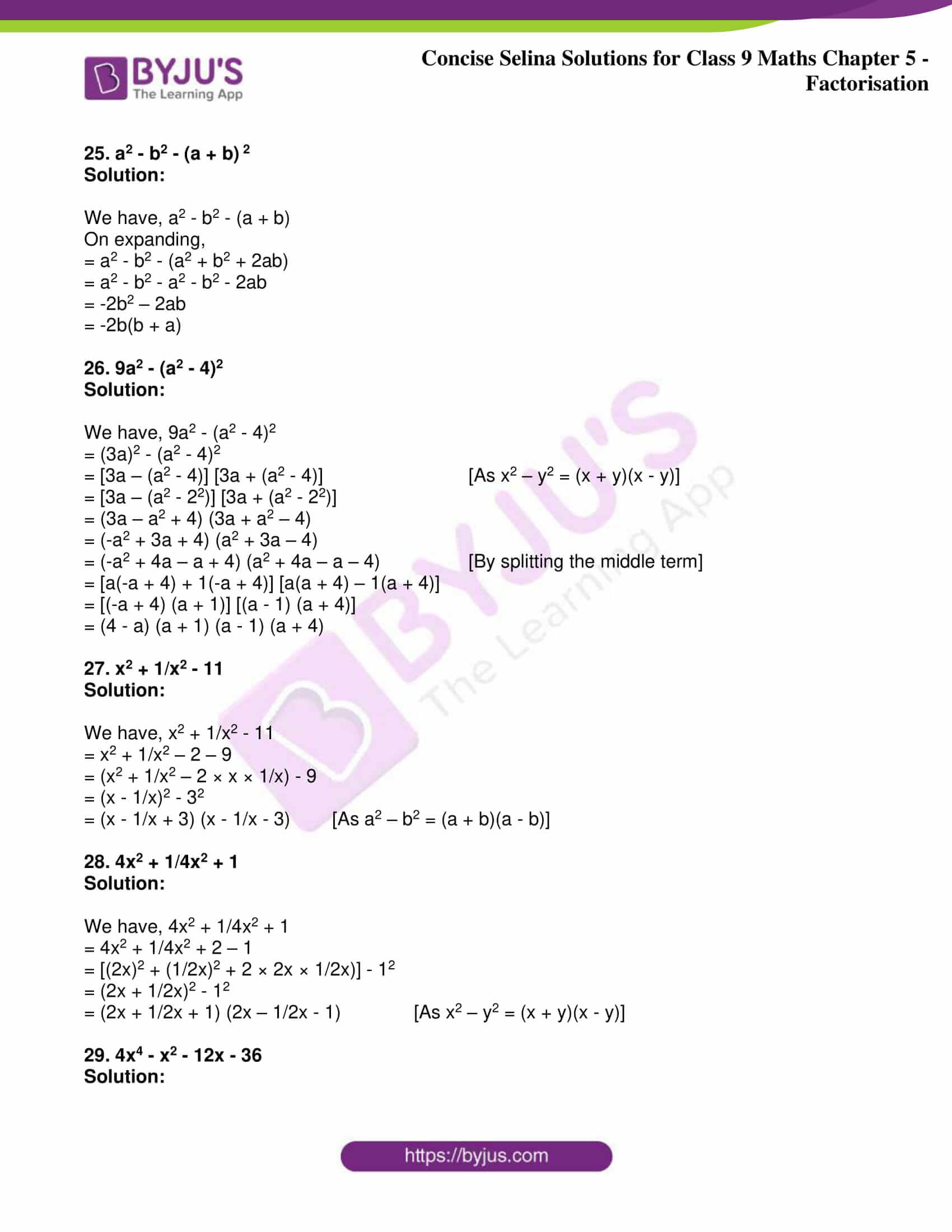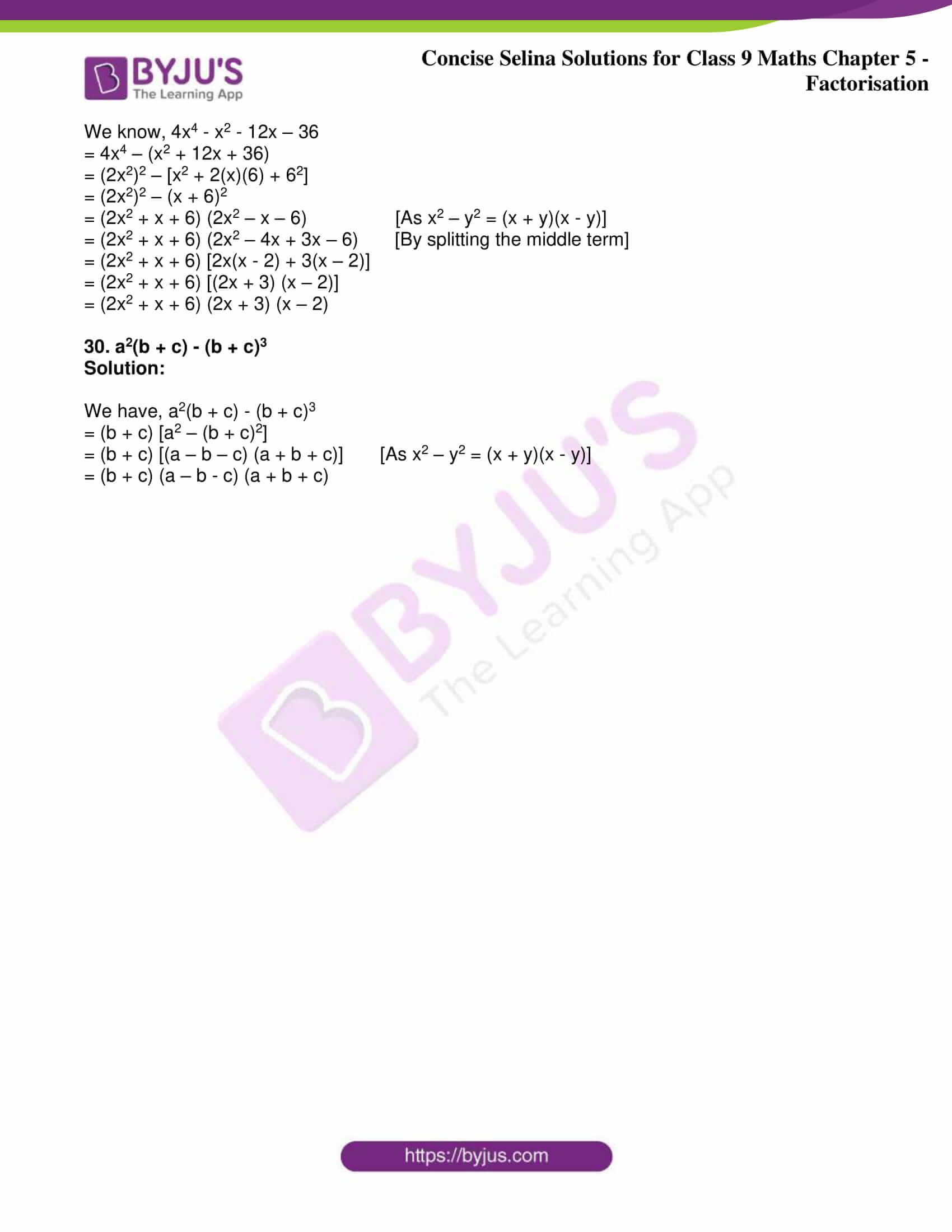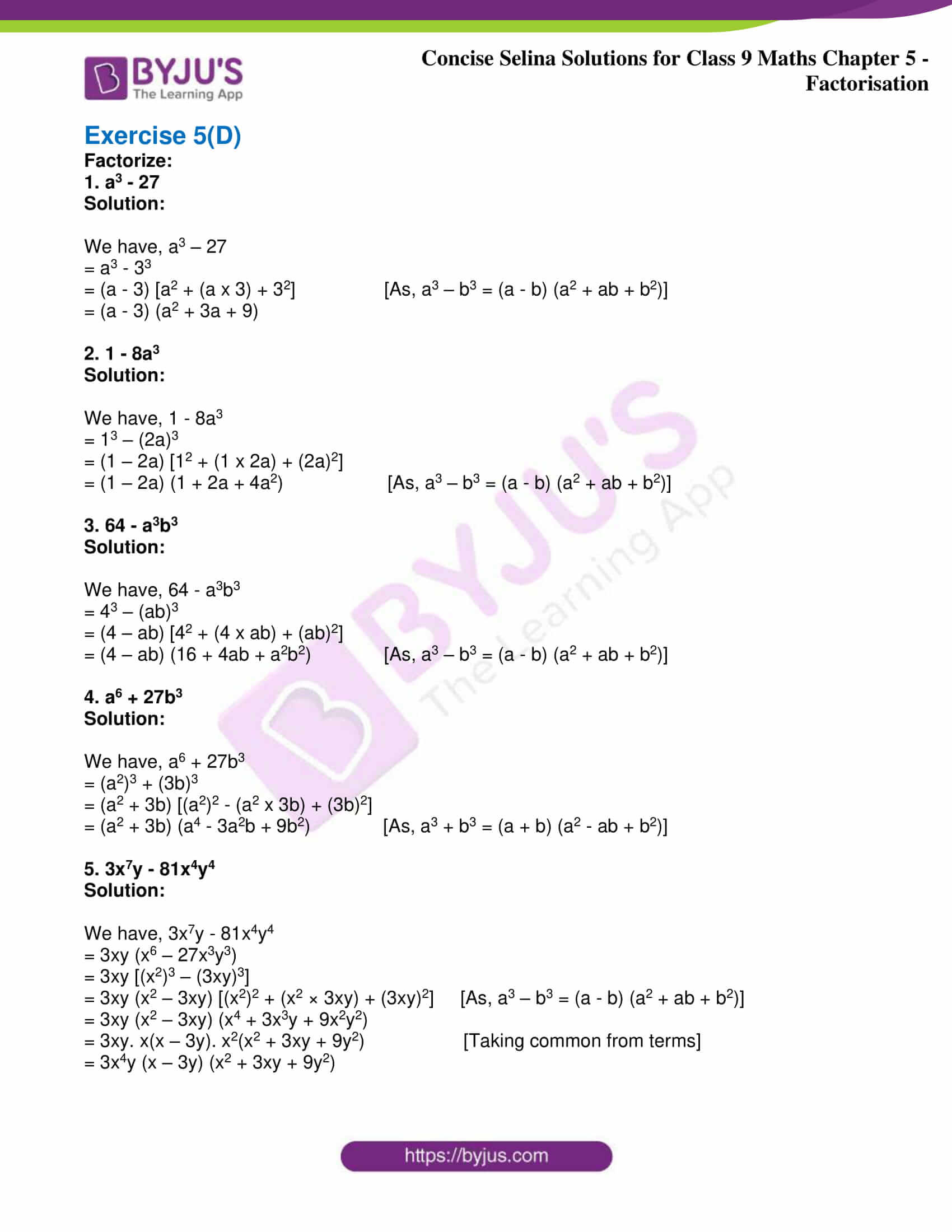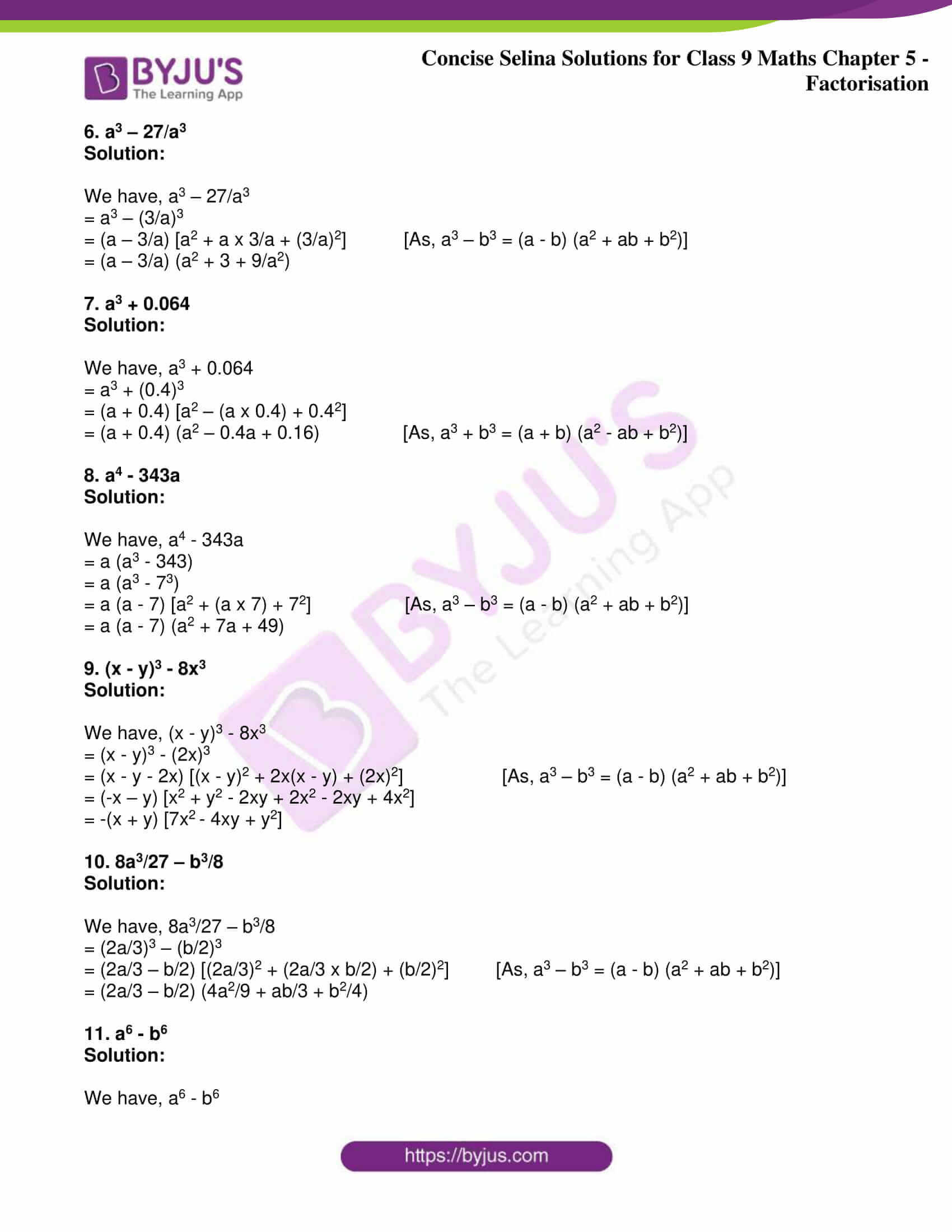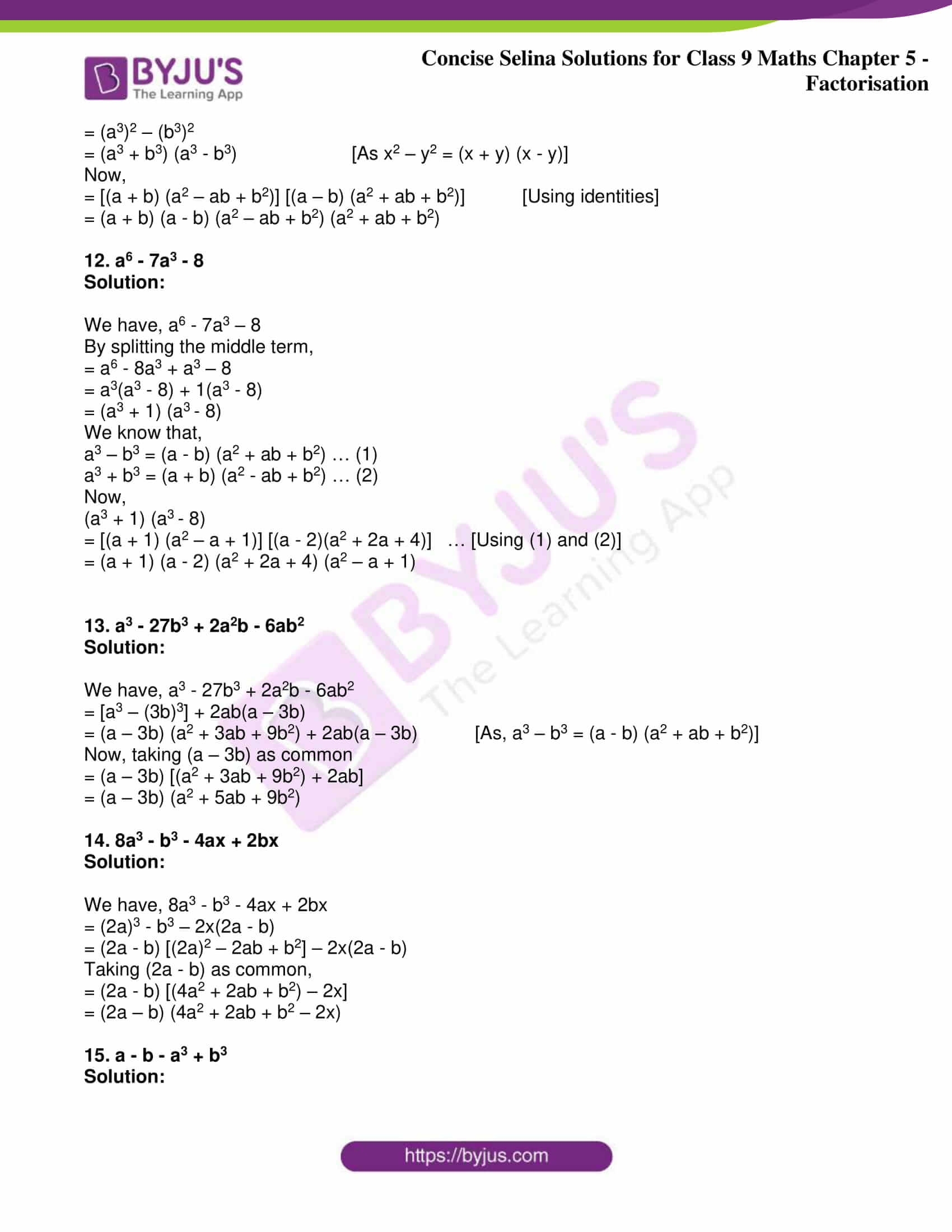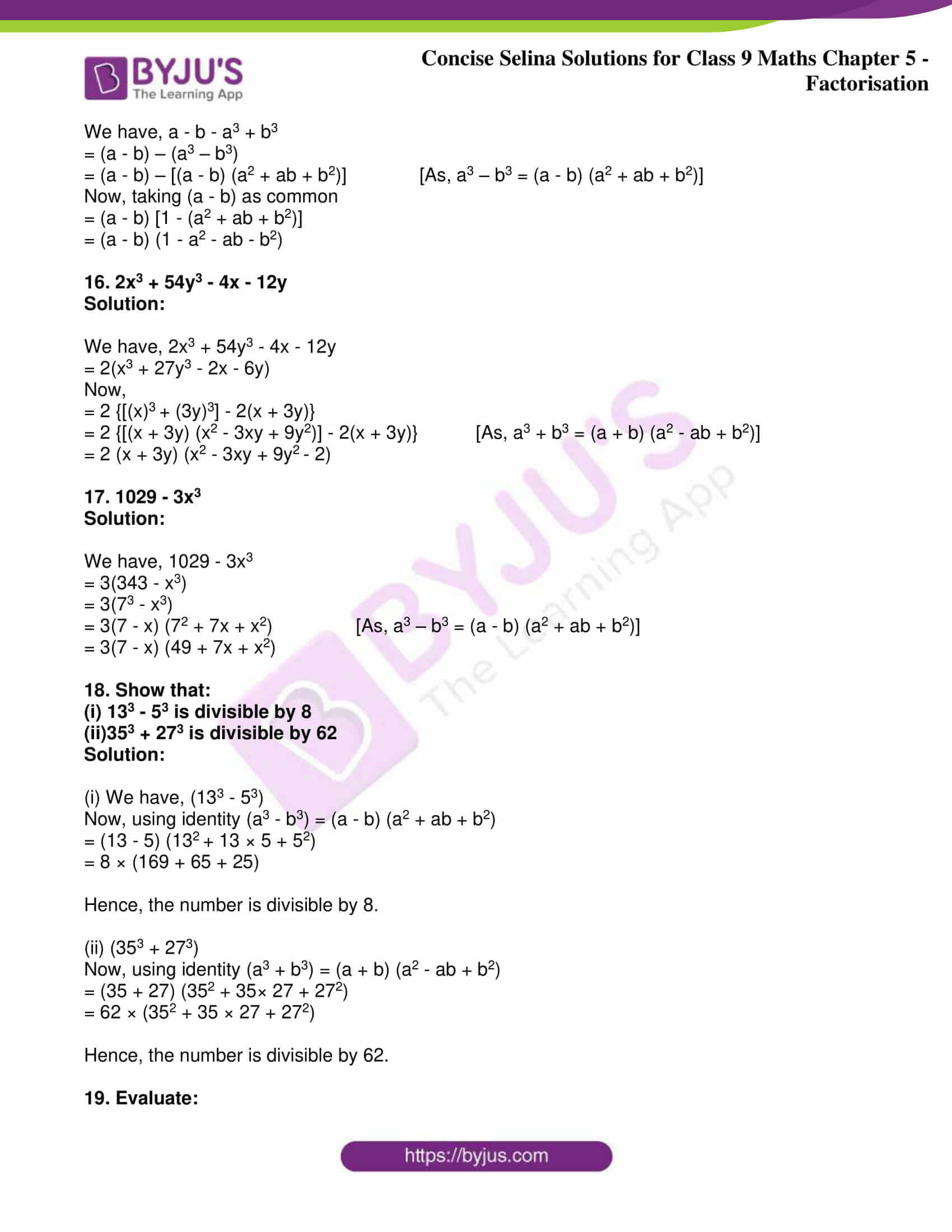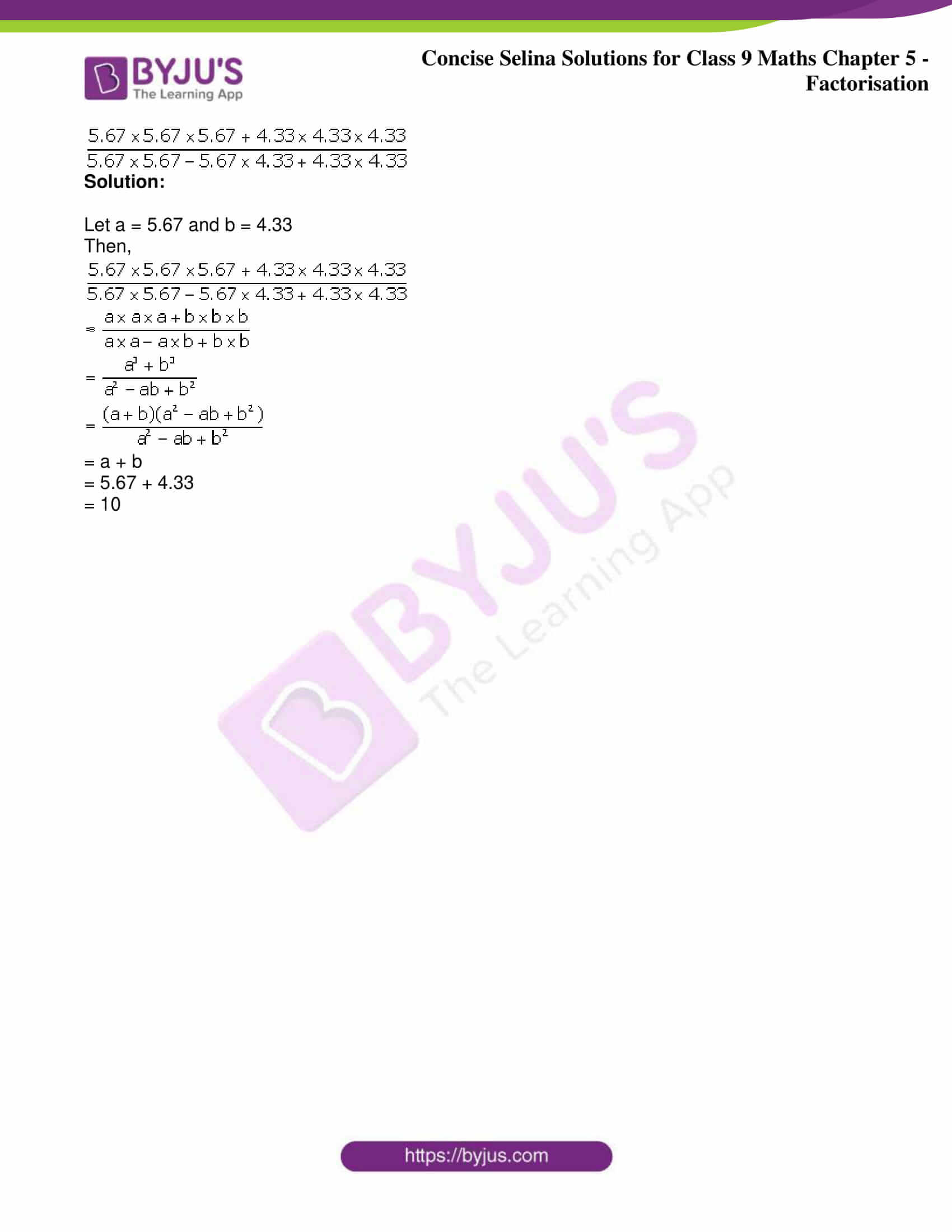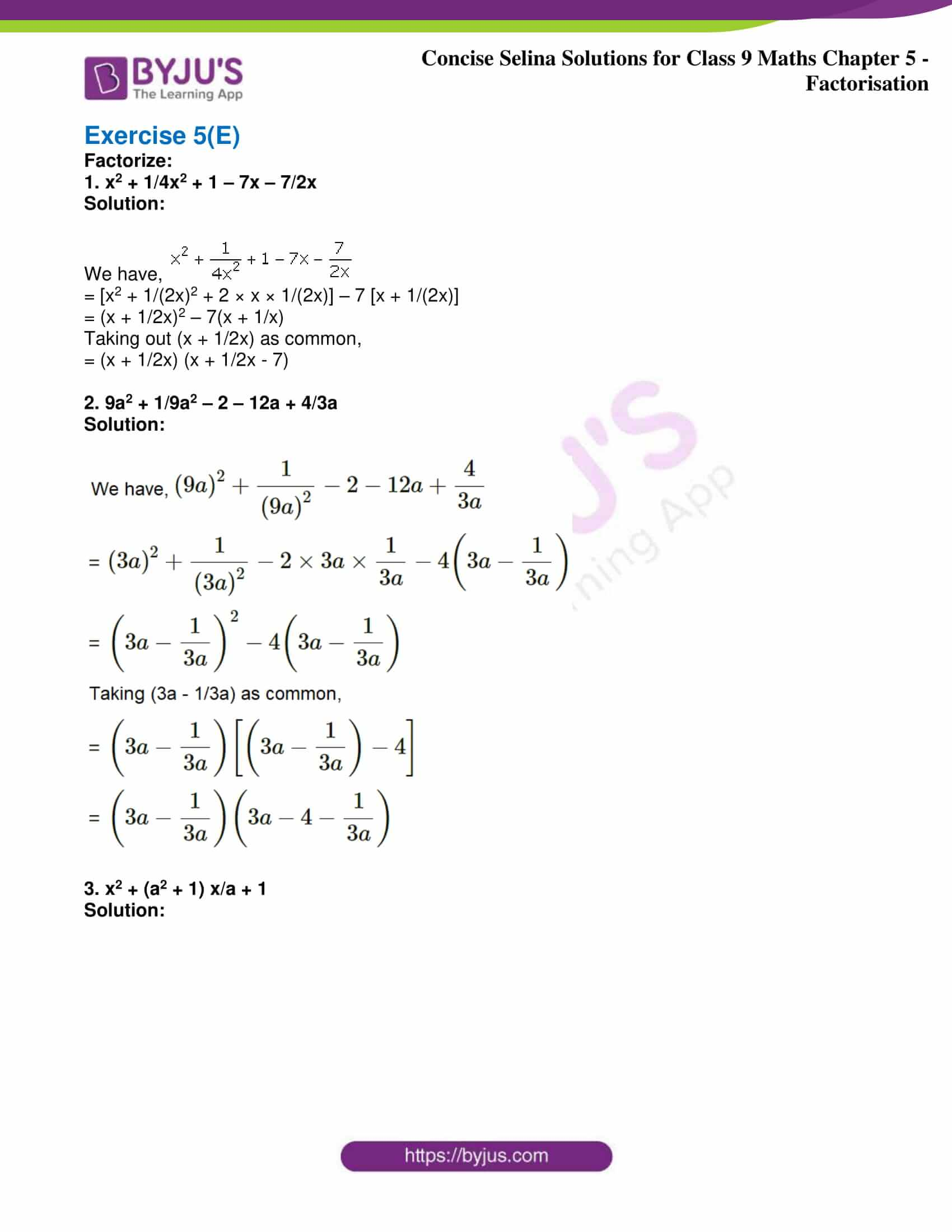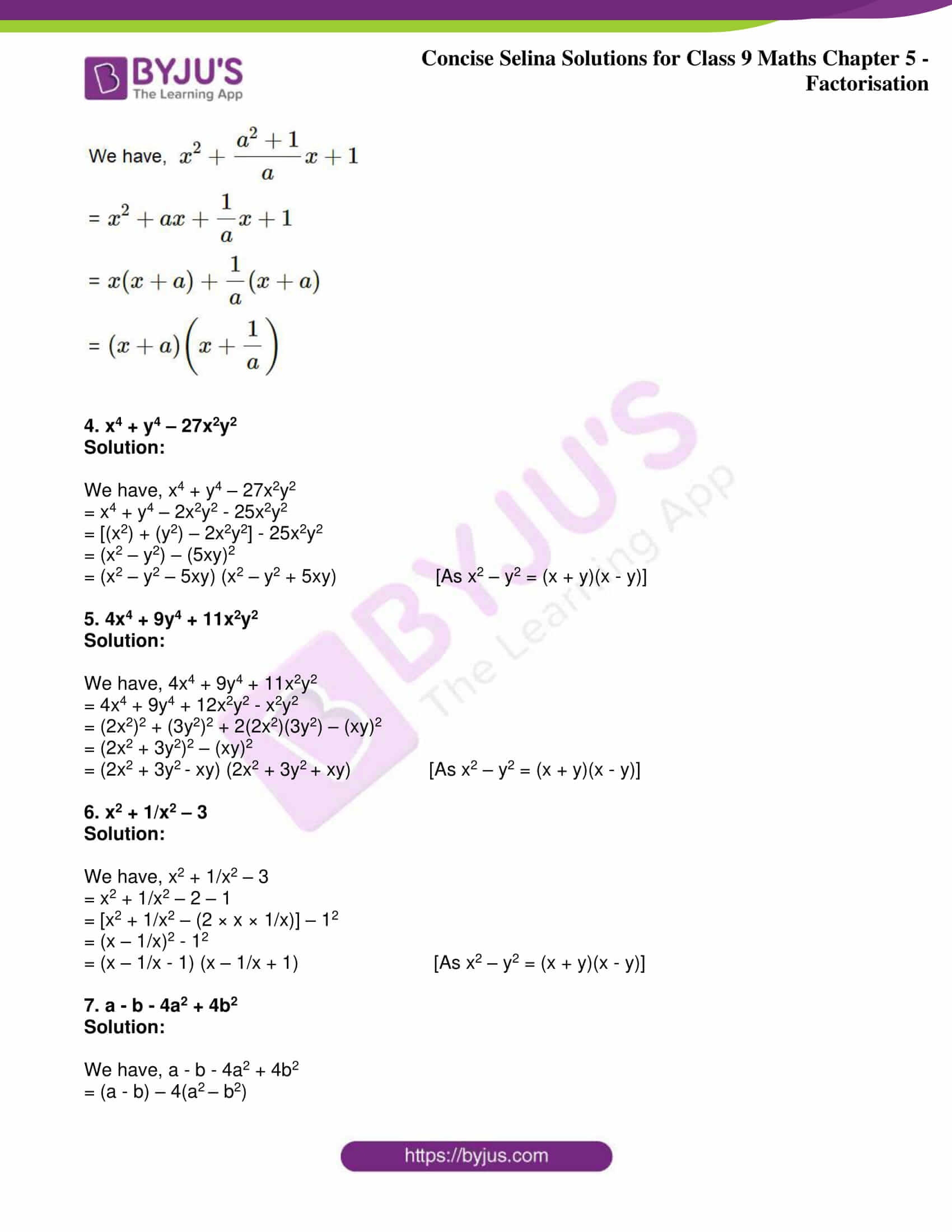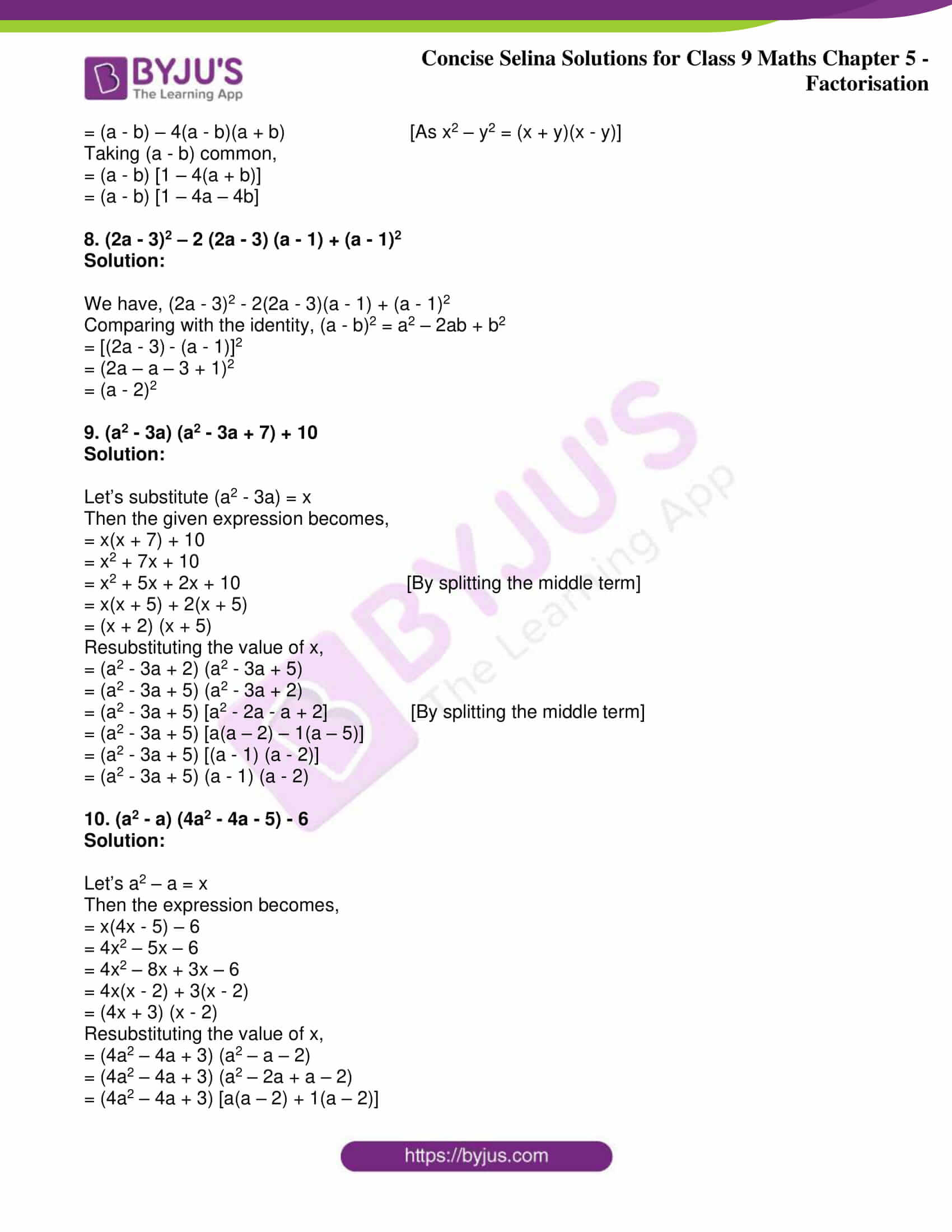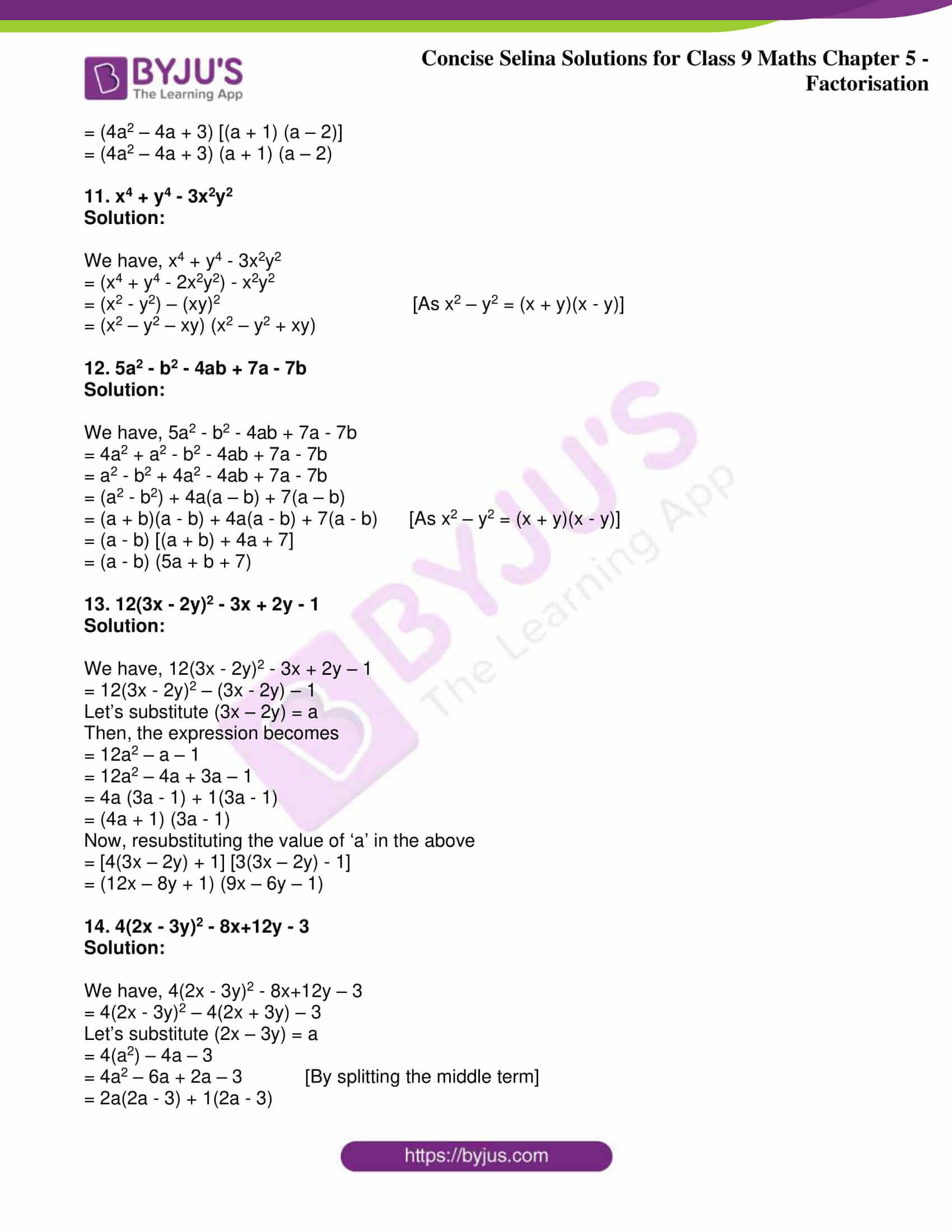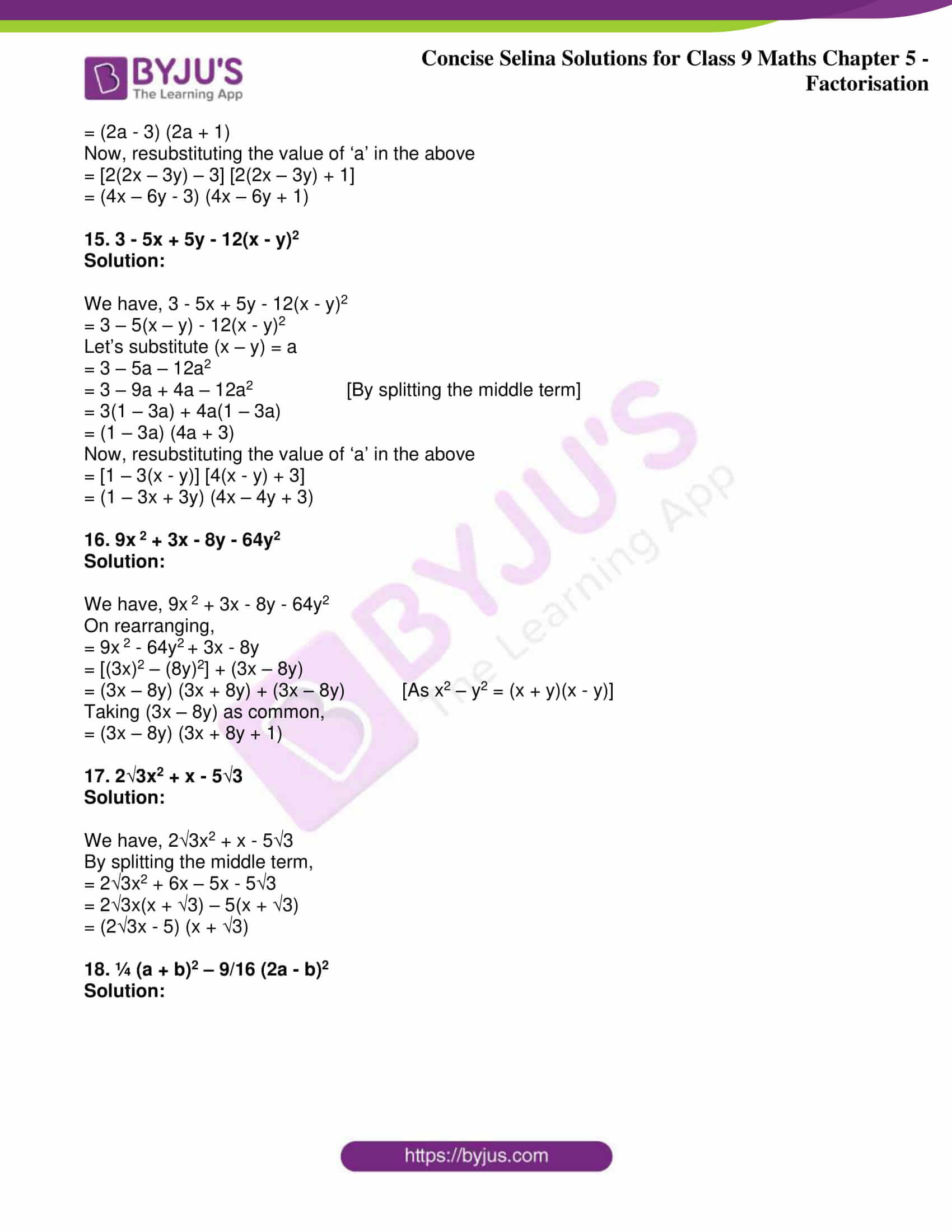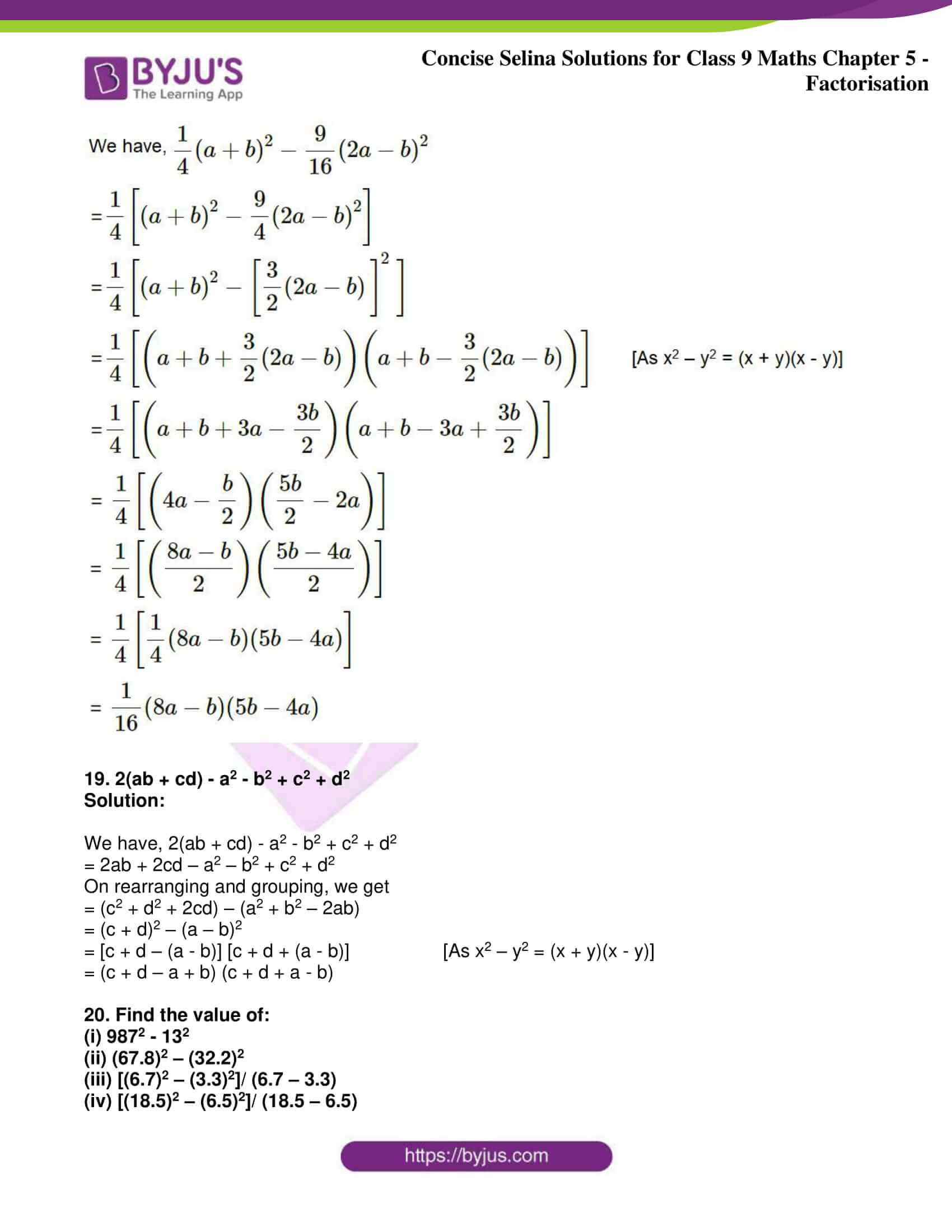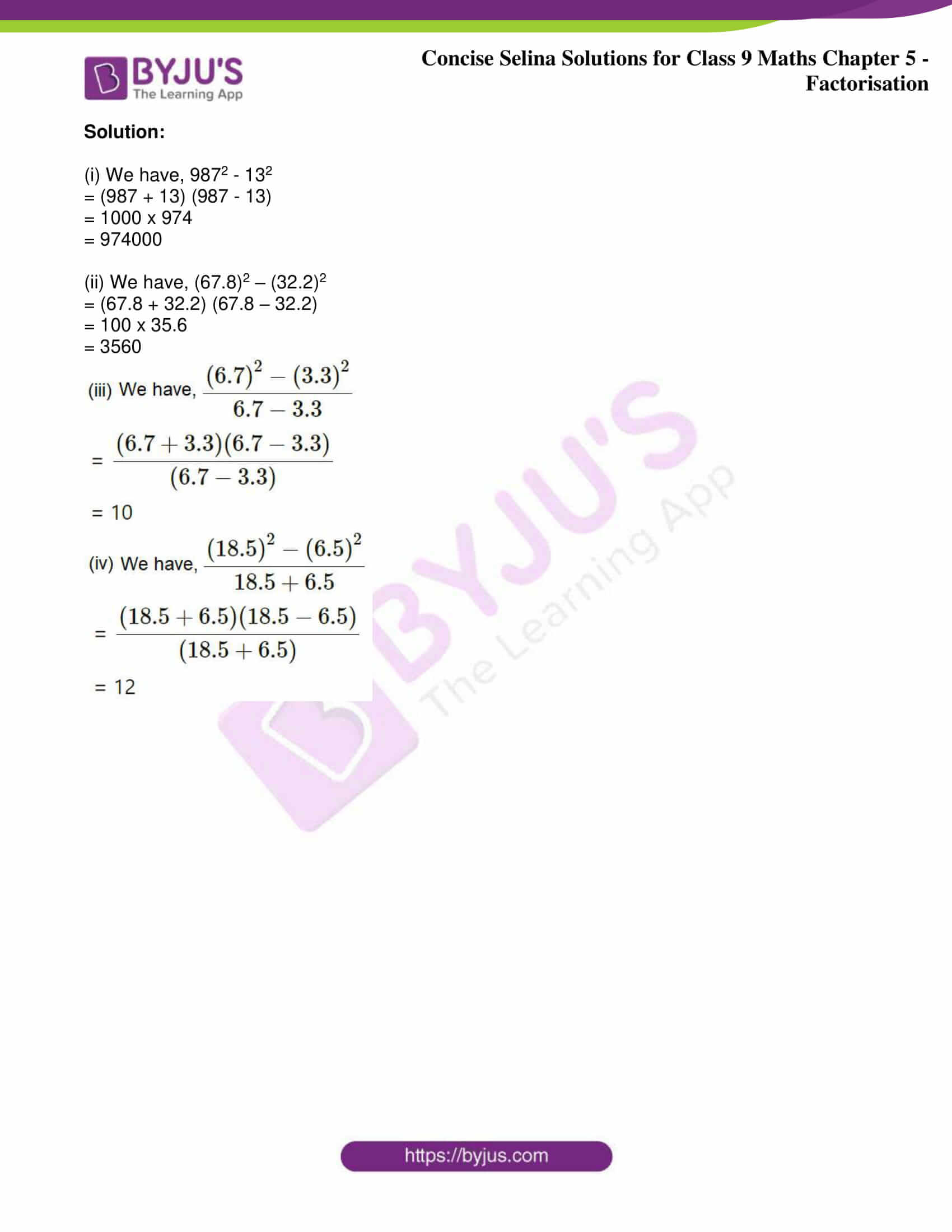Exercise 5(A)

Factorise by taking out the common factors:

1. 2(2x – 5y) (3x + 4y) – 6(2x – 5y) (x – y)

Solution:

Identifying and taking (2x – 5y) common from both the terms, we have

= (2x – 5y) [2(3x + 4y) – 6(x – y)]

= (2x – 5y) (6x + 8y – 6x + 6y)

= (2x – 5y) (8y + 6y)

= (2x – 5y) (14y)

= (2x – 5y)14y

2. xy(3x– 2y2) – yz(2y– 3x2) + zx(15x– 10y2)

Solution:

We have, xy(3x– 2y2) – yz(2y– 3x2) + zx(15x– 10y2)

Changing signs to arrive at a common term

So,

= xy(3x– 2y2) + yz(3x– 2y2) + zx(15x– 10y2)

= xy(3x– 2y2) + yz(3x– 2y2) + 5zx(3x– 2y2)

= (3x2 – 2y2) (xy + yz + 5zx)

3. ab(a+ b– c2) – bc(c– a– b2) + ca(a+ b– c2)

Solution:

We have, ab(a+ b– c2) – bc(c– a– b2) + ca(a+ b– c2)

Changing signs to arrive at a common term

So,

= ab(a+ b– c2) + bc(a+ b– c2) + ca(a+ b– c2)

= (a+ b– c2) (ab + bc + ca)

4. 2x(a – b) + 3y(5a – 5b) + 4z(2b – 2a)

Solution:

We have, 2x(a – b) + 3y(5a – 5b) + 4z(2b – 2a)

Taking common factors, we get

= 2x(a – b) + 15y(a – b) – 8z(a – b)

= (a – b) (2x + 15y – 8z)

Factorize by the grouping method:

5. a3 + a – 3a2 – 3

Solution:

We have, a3 + a – 3a2 – 3

Grouping to arrive at a common term

= a (a2 + 1) – 3(a2 + 1)

Taking common, we get

= (a2 + 1) (a -3)

6. 16(a + b)2 – 4a – 4b

Solution:

We have, 16 (a + b)2 – 4a – 4b

Grouping to arrive at a common term

= 16(a + b)2 – 4 (a + b)

Taking common, we get

= 4(a + b) [4 (a + b) – 1]

= 4(a + b) (4a + 4b – 1)

7. Factorize by the grouping method:

a4 – 2a3 – 4a + 8

Solution:

We have, a4 – 2a3 – 4a + 8

Grouping to arrive at a common term

= a3(a – 2) – 4(a – 2)

Taking common, we get

= (a3 – 4) (a – 2)

8. ab – 2b + a2 – 2a

Solution:

We have, ab – 2b + a2 – 2a

Grouping to arrive at a common term

= b(a – 2) + a(a – 2)

Taking common, we get

= (b + a) (a – 2)

9. ab(x2 + 1) + x(a2 + b2)

Solution:

We have, ab(x2 + 1) + x(a2 + b2)

On expanding,

= abx2 + ab + a2x + b2x

Now, grouping to arrive at a common term

= abx2 + a2x + b2x + ab

= ax(bx + a) + b(bx + a)

Taking common, we get

= (ax + b) (bx + a)

10. a2 + b – ab – a

Solution:

We have, a2 + b – ab – a

Grouping to arrive at a common term

= a2 – a + b – ab

= a(a – 1) – b(-1 + a)

= a(a – 1) – b(a – 1)

Taking common, we get

= (a – b) (a – 1)

11. (ax + by)2 + (bx – ay)2

Solution:

We have, (ax + by)2 + (bx – ay)2

On expanding,

= a2x2 + b2y2 + 2abxy + b2x2 + a2y2 – 2abxy

= a2x2 + b2y2 + b2x2 + a2y2

Rearranging terms, we get

= a2x2 + b2x2 + a2y2 + b2y2

Taking common, we get

= x2(a2 + b2) + y2(a2 + b2)

= (x2 + y2) (a2 + b2)

12. a2x2 + (ax2 + 1)x + a

Solution:

We have, a2x2 + (ax2 + 1)x + a

Regrouping the terms, we have

= a2x2 + a + (ax2 + 1)x

= a(ax2 + 1) + x(ax2 + 1)

Taking common, we get

= (ax2 + 1) (a + x)

13. (2a – b)2 – 10a + 5b

Solution:

We have, (2a – b)2 – 10a + 5b

Taking common,

= (2a – b)2 – 5(2a – b)

Now,

= (2a – b) [(2a – b) – 5]

= (2a – b) (2a – b – 5)

14. a(a – 4) – a + 4

Solution:

We have, a(a – 4) – a + 4

By grouping, we get

= a(a – 4) -1(a – 4)

Now, taking the common term

= (a – 4) (a – 1)

15. y2 – (a + b) y + ab

Solution:

We have, y2 – (a + b) y + ab

On expanding,

= y2 – ay – by + ab

= (y2 – ay) – by + ab

Taking ‘y’ and ‘b’ common from the group, we get

= y(y – a) – b(y – a)

= (y – a) (y – b)

16. a2 + 1/a2 – 2 – 3a + 3/a
Solution:

We have, a2 + 1/a2 – 2 – 3a + 3/a

On grouping terms, we get

= (a2 – 2 + 1/a2) – 3a + 3/a

= [a2 – (2 x a x 1/a) + 1/a2] – 3(a – 1/a)

= (a – 1/a)2 – 3(a – 1/a) {Since, (x – y)2 = x2 – 2xy + y2}

Taking (a – 1/a) as common, we get

= (a – 1/a) [(a – 1/a) – 3]

= (a – 1/a) (a – 1/a – 3)

17. x2 + y2 + x + y + 2xy

Solution:

We have, x2 + y2 + x + y + 2xy

On rearranging terms, we get

= (x2 + y2 + 2xy) + (x + y) {Since, (x + y)2 = x+ 2xy + y2}

Now,

= (x + y)+ (x + y)

= (x + y)(x + y + 1)

18. a2 + 4b2 – 3a + 6b – 4ab

Solution:

We have, a2 + 4b2 – 3a + 6b – 4ab

On rearranging terms, we get

= a2 + 4b2 – 4ab – 3a + 6b

Now,

= a2 + (2b)2 – 2 × a × (2b) – 3(a – 2b) {Since, (a – b)2 = a2 – 2ab + b2}

= (a – 2b)– 3(a – 2b)

= (a – 2b) [(a – 2b)- 3]

= (a – 2b) (a – 2b – 3)

19. m (x – 3y)2 + n (3y – x) + 5x – 15y

Solution:

We have, m (x – 3y)2 + n (3y – x) + 5x – 15y

Now,

Taking (x – 3y) common from all the three terms, we get

= m(x – 3y)2 – n(x – 3y) + 5(x – 3y)

= (x – 3y) [m(x – 3y) – n + 5]

= (x – 3y) (mx – 3my – n + 5)

20. x(6x – 5y) – 4(6x – 5y)2

Solution:

We have, x(6x – 5y) – 4(6x – 5y)2

Now,

Taking (6x – 5y) common from the three terms, we get

= (6x – 5y) [x – 4(6x – 5y)]

= (6x – 5y) (x – 24x + 20y)

= (6x – 5y) (-23x + 20y)

= (6x – 5y) (20y – 23x)

Exercise 5(B)

Factorize:

1. a2 + 10a + 24

Solution:

We have, a2 + 10a + 24

By splitting the middle term, we get

= a2 + 6a + 4a + 24

= a(a + 6) + 4(a + 6)

= (a + 4) (a + 6)

2. a2 – 3a – 40

Solution:

We have, a2 – 3a – 40

By splitting the middle term, we get

= a2 – 8a + 5a – 40

= a(a – 8) + 5(a – 8)

= (a + 5) (a – 8)

3. 1 – 2a – 3a2

Solution:

We have, 1 – 2a – 3a2

By splitting the middle term, we get

= 1 – 3a + a – 3a2

= 1(1 – 3a) + a(1 – 3a)

= (1 + a) (1 – 3a)

4. x2 – 3ax – 88a2

Solution:

We have, x2 – 3ax – 88a2

By splitting the middle term, we get

= x2 – 11ax + 8ax – 88a2

= x(x – 11a) + 8a(x – 11a)

= (x + 8a) (x – 11a)

5. 6a2 – a – 15

Solution:

We have, 6a2 – a – 15

By splitting the middle term, we get

= 6a2 + 9a – 10a – 15

= 3a(2a + 3) – 5(2a + 3)

= (3a – 5) (2a + 3)

6. 24a3 + 37a2 – 5a

Solution:

We have, 24a3 + 37a2 – 5a

Taking ‘a’ common from all

= a (24a2 + 37a – 5)

= a (24a2 + 40a – 3a – 5) {By splitting the middle term}

= a [8a(3a + 5) – 1(3a + 5)]

= a [(8a – 1) (3a + 5)]

= a (8a – 1) (3a + 5)

7. a(3a – 2) – 1

Solution:

We have, a(3a – 2) – 1

On expanding,

= 3a2 – 2a – 1

By splitting the middle term, we get

= 3a2 – 3a + a – 1

= 3a(a – 1) + 1(a – 1)

= (3a + 1) (a – 1)

8. a2b2 + 8ab – 9

Solution:

We have, a2b2 + 8ab – 9

By splitting the middle term, we get

= a2b2 + 9ab – ab – 9

= ab(ab + 9) – 1(ab + 9)

= (ab – 1) (ab + 9)

9. 3 – a (4 + 7a)

Solution:

We have, 3 – a (4 + 7a)

On expanding,

= 3 – 4a – 7a2

By splitting the middle term, we get

= 3 + 3a – 7a – 7a2

= 3(1 + a) – 7a(1 + a)

= (1 + a) (3 – 7a)

10. (2a + b)2 – 6a – 3b – 4

Solution:

We have, (2a + b)2 – 6a – 3b – 4

= (2a + b)2 – 3(2a + b) – 4

Let’s assume that (2a + b) = x

So, the expression becomes

= x2 – 3x – 4

By splitting the middle term, we get

= x2 – 4x + x – 4

= x(x – 4) + 1(x – 4)

= (x – 4) (x + 1)

Resubstituting the value of x, we get

= (2a + b – 4) (2a + b + 1)

11. 1 – 2 (a+ b) – 3 (a + b)2

Solution:

We have, 1 – 2 (a+ b) – 3 (a + b)2

Let’s assume (a + b) = x

Then, the expression becomes

= 1 – 2x – 3x2

By splitting the middle term, we get

= 1 – 3x + x – 3x2

= 1(1 – 3x) + x(1 – 3x)

= (1 – 3x) (1 + x)

Resubstituting the value of x, we get

= [1 – 3(a + b)] [1 + (a + b)]

= (1 – 3a – 3b) (1 + a + b)

12. 3a2 – 1 – 2a

Solution:

We have, 3a2 – 1 – 2a

Rearranging,

= 3a2 – 2a – 1

By splitting the middle term, we get

= 3a2 – 3a + a – 1

= 3a(a – 1) + 1(a – 1)

= (3a + 1) (a – 1)

13. x2 + 3x + 2 + ax + 2a

Solution:

We have, x2 + 3x + 2 + ax + 2a

By splitting the middle term, we get

= (x2 + 2x + x + 2) + ax + 2a

= x(x + 2) + 1(x + 2) + a(x + 2)

= (x + 2) (x + a + 1)

14. (3x – 2y)2 + 3 (3x – 2y) – 10

Solution:

We know, (3x – 2y)2 + 3 (3x – 2y) – 10

Let’s assume that (3x – 2y) = a

So, the expression becomes

= a2 + 3a – 10

By splitting the middle term, we get

= a2 + 5a – 2a – 10

= a(a + 5) – 2(a + 5)

= (a – 2) (a + 5)

= (3x – 2y + 5) (3x – 2y – 2)

15. 5 – (3a2 – 2a) (6 – 3a2 + 2a)

Solution:

Given, 5 – (3a2 – 2a) (6 – 3a2 + 2a)

= 5 – (3a2 – 2a) [6 – (3a2 – 2a)]

Let’s substitute (3a2 – 2a) = x

And, the expression becomes,

= 5 – x(6 – x)

= 5 – 6x – x2

= 5 – 5x – x – x2

= 5(1 – x) – x(1 – x)

= (1 – x) (5 – x)

= (x – 1) (x – 5)

= (3a2 – 2a – 1) (3a2 – 2a – 5)

Now,

= (3a2 – 3a + a – 1) (3a2 + 3a – 5a – 5) {By splitting the middle term}

= [3a(a – 1) + 1(a – 1)] [3a(a + 1) – 5(a + 1)]

= [(3a + 1) (a – 1)] [(3a – 5) (a + 1)]

= (3a + 1) (3a – 5) (a + 1)(a – 1)

16. 1/35 + 12a/35 + a2

Solution:

We have, 1/35 + 12a/35 + a2

Taking common,

= 1/35 (1 + 12a + 35a2)

= 1/35 (35a2 + 12a + 1)

= 1/35 (35a2 + 7a + 5a + 1) {By splitting the middle term}

= 1/35 [7a(5a + 1) + 1(5a + 1)]

= 1/35 [(7a + 1) (5a + 1)]

= [(7a + 1) (5a + 1)]/ 35

17. (x– 3x) (x– 3x – 1) – 20.

Solution:

We have, (x– 3x) (x– 3x – 1) – 20

= (x2 – 3x)[(x2 – 3x) – 1] – 20

Let’s

= a[a – 1] – 20 ….(Taking x2 – 3x = a)

= a2 – a – 20

= a2 – 5a + 4a – 20

= a(a – 5) + 4(a – 5)

= (a – 5)(a + 4)

= (x2 – 3x – 5)(x2 – 3x + 4)

18. Find each trinomial (quadratic expression), given below, find whether it is factorisable or not. Factorise, if possible.

(i) x2 – 3x – 54

(ii) 2x2 – 7x – 15

(iii) 2x2 + 2x – 75

(iv) 3x2 + 4x – 10

(v) x(2x – 1) – 1

Solution:

(i) Given, x2 – 3x – 54

On comparing with the general form ax2 + bx + c, we get

a = 1, b = -3 and c = -54

So, b2 – 4ac = (-3)2 – 4(1)(-54) = 9 + 216 = 225

225 is a perfect square

Thus, x2 – 3x – 54 is factorisable

Now,

x2 – 3x – 54 = x2 – 9x + 6x – 54

= x(x – 9) + 6(x – 9)

= (x + 6) (x – 9)

(ii) Given, 2x2 – 7x – 15

On comparing with the general form ax2 + bx + c, we get

a = 2, b = -7 and c = -15

So, b2 – 4ac = (-7)2 – 4(2)(-15) = 49 + 120 = 169

169 is a perfect square

Thus, 2x2 – 7x – 15 is factorisable

Now,

2x2 – 7x – 15 = 2x2 – 10x + 3x – 15

= 2x(x – 5) + 3(x – 5)

= (2x + 3) (x – 5)

(iii) Given, 2x2 + 2x – 75

On comparing with the general form ax2 + bx + c, we get

a = 2, b = 2 and c = -75

So, b2 – 4ac = (2)2 – 4(2)(-75) = 4 + 600 = 604

604 is not a perfect square

Thus, 2x2 + 2x – 75 is not factorizable

(iv) Given, 3x2 + 4x – 10

On comparing with the general form ax2 + bx + c, we get

a = 3, b = 4 and c = -10

So, b2 – 4ac = (4)2 – 4(3)(-10) = 16 + 120 = 136

136 is a not perfect square

Thus, 3x2 + 4x – 10 is not factorizable

(v) Given, x(2x – 1) – 1

= 2x2 – x – 1

On comparing with the general form ax2 + bx + c, we get

a = 2, b = -1 and c = -1

So, b2 – 4ac = (-1)2 – 4(2)(-1) = 1 + 8 = 9

9 is a perfect square

Thus, x(2x – 1) – 1 is factorisable

Now,

x(2x – 1) – 1 = 2x2 – x – 1

= 2x2 – 2x + x – 1

= 2x(x – 1) + 1(x – 1)

= (2x + 1) (x – 1)

19. Factorise:

(i) 4√3x2 + 5x – 2√3

(ii) 7√2x2 – 10x – 4√2

Solution:

(i) We have, 4√3x2 + 5x – 2√3

By splitting the middle term, we get

= 4√3x2 + 8x – 3x – 2√3

= 4x(√3x + 2) – √3(√3x + 2)

= (4x – √3) (√3x + 2)

(ii) We have, 7√2x2 – 10x – 4√2

By splitting the middle term, we get

= 7√2x2 – 14x + 4x – 4√2

= 7√2x(x – √2) + 4(x – √2)

= (7√2x + 4) (x – √2)

20. Give possible expressions for the length and the breadth of the rectangle whose area is 12x– 35x + 25.

Solution:

We have, 12x– 35x + 25

By splitting the middle term, we get

= 12x2 – 20x – 15x + 25

= 4x(3x – 5) – 5(3x – 5)

= (3x – 5) (4x – 5)

Hence,

Length = (3x – 5) and breadth = (4x – 5) or,

Length = (4x – 5) and breadth = (3x – 5)

Exercise 5(C)

Factorize:

1. 25a2 – 9b2

Solution:

We have, 25a2 – 9b2

= (5a)2 – (3b)2

= (5a + 3b) (5a – 3b) [As x2 – y2 = (x + y)(x – y)]

2. a2 – (2a + 3b)2

Solution:

We have, a2 – (2a + 3b)2

= [a – (2a + 3b)] [a + (2a + 3b)] [As x2 – y2 = (x + y)(x – y)]

= (a – 2a – 3b) (a + 2a + 3b)

= (-a – 3b) (3a + 3b)

= -3(a + 3b) (a + b)

3. a2 – 81(b-c)2

Solution:

We have, a2 – 81(b-c)2

= a2 – [9(b – c)]2

= [a – 9(b – c)] [a + 9(b – c)]] [As x2 – y2 = (x + y)(x – y)]

= (a – 9b + 9c) (a + 9b – 9c)

4. 25(2a – b)2 – 81b2

Solution:

We have, 25(2a – b)2 – 81b2

= [5(2a – b)]2 – (9b)2

= [5(2a – b) – 9b] [5(2a – b) + 9b] [As x2 – y2 = (x + y)(x – y)]

= (10a – 5b – 9b) (10a – 5b + 9b)

= (10a – 14b) (10a + 4b)

= 2(5a – 7b). 2(5a + 2b)

= 2(5a – 7b) (5a + 2b)

5. 50a3 – 2a

Solution:

We have, 50a3 – 2a

= 2a(25a2 – 1)

= 2a[(5a)– 12]

= 2a(5a – 1)(5a + 1) [As x2 – y2 = (x + y)(x – y)]

6. 4a2b – 9b3

Solution:

We have, 4a2b – 9b3

= b(4a2 – 9b2)

= b[(2a)2 – (3b)2]

= b[(2a + 3b) (2a – 3b)] [As x2 – y2 = (x + y)(x – y)]

= b(2a + 3b)(2a – 3b)

7. 3a5 – 108a3

Solution:

We have, 3a5 – 108a3

= 3a3(a2 – 36)

= 3a3(a2 – 62)

= 3a3(a – 6) (a + 6) [As x2 – y2 = (x + y)(x – y)]

8. 9(a – 2)2 – 16(a + 2)2

Solution:

We have, 9(a – 2)2 – 16(a + 2)2

= [3(a – 2)]2 – [4(a + 2)]2

= [3(a – 2) – 4(a + 2)] [3(a – 2) + 4(a + 2)] [As x2 – y2 = (x + y)(x – y)]

= [3a – 6 – 4a – 8] [3a – 6 + 4a + 8]

= [-a – 14] [7a + 2]

= -(a + 14) (7a + 2)

9. a4 – 1

Solution:

We have, a4 – 1

= (a2)2 – 12

= (a2 – 1) (a2 + 1) [As x2 – y2 = (x + y)(x – y)]

= [(a – 1)(a + 1)] (a2 + 1)

= (a – 1)(a + 1)(a2 + 1)

10. a3 + 2a2 – a – 2

Solution:

We have, a3 + 2a2 – a – 2

= a2(a + 2) – 1(a + 2)

= (a2 – 1) (a + 2)

= (a – 1) (a + 1) (a + 2) [As x2 – y2 = (x + y)(x – y)]

11. (a + b)3 – a – b

Solution:

We have, (a + b)3 – a – b

= (a + b)3 – (a + b)

= (a + b) [(a + b)2 – 1]

= (a + b) [(a + b – 1) (a + b + 1)] [As x2 – y2 = (x + y)(x – y)]

= (a + b) (a + b -1) (a + b + 1)

12. a(a – 1) – b(b – 1)

Solution:

We have, a(a – 1) – b(b – 1)

= a2 – a – b2 + b

= (a2 – b2) – (a – b)

= (a + b) (a – b) – (a – b) [As x2 – y2 = (x + y)(x – y)]

= (a – b) [(a + b) – 1]

= (a – b) (a + b – 1)

13. 4a2 – (4b2 + 4bc + c2)

Solution:

We know, 4a2 – (4b2 + 4bc + c2)

= (2a)2 – [(2b)2 + 2(2b)(c) + c2]

= (2a)2 – (2b + c)2

= (2a – 2b – c) (2a + 2b + c) [As x2 – y2 = (x + y)(x – y)]

14. 4a2 – 49b2 + 2a – 7b

Solution:

We know, 4a2 – 49b2 + 2a – 7b

= (2a)2 – (7b)2 + (2a – 7b)

= [(2a – 7b) (2a + 7b)] + (2a – 7b) [As x2 – y2 = (x + y)(x – y)]

= (2a – 7b) [(2a + 7b) + 1]

= (2a – 7b) (2a + 7b + 1)

15. 9a2 + 3a – 8b – 64b2

Solution:

We have, 9a2 + 3a – 8b – 64b2

= 9a2 – 64b2 + 3a – 8b

= (3a)2 – (8b)2 + (3a – 8b)

= [(3a – 8b) (3a + 8b)] + (3a – 8b) [As x2 – y2 = (x + y)(x – y)]

= (3a – 8b) [(3a + 8b) + 1]

= (3a – 8b) (3a + 8b + 1)

16. 4a2 – 12a + 9 – 49b2

Solution:

We have, 4a2 – 12a + 9 – 49b2

= [(2a)2 – 2(2a)(3) + 32] – (7b)2

= (2a – 3)2 – (7b)2

= (2a – 7b – 3) (2a + 7b – 3) [As x2 – y2 = (x + y)(x – y)]

17. 4xy – x2 – 4y2 + z2

Solution:

We have, 4xy – x2 – 4y2 + z2

On rearranging,

= z2 – x2 – 4y2 + 4xy

= z2 – (x2 + 4y2 – 4xy)

= z2 – (x – 2y)2

= (z – x + 2y) (z + x – 2y) [As x2 – y2 = (x + y)(x – y)]

18. a2 + b2 – c2 – d2 + 2ab – 2cd

Solution:

We have, a2 + b2 – c2 – d2 + 2ab – 2cd

On rearranging,

= a2 + 2ab + b2 – c2 – d2 – 2cd

= (a2 + 2ab + b2) – (c2 + d2 + 2cd)

= (a + b)2 – (c + d)2

= (a + b + c + d) (a + b – c – d) [As x2 – y2 = (x + y)(x – y)]

19. 4x2 – 12ax – y2 – z2 – 2yz + 9a2

Solution:

We have, 4x2 – 12ax – y2 – z2 – 2yz + 9a2

On rearranging,

= 4x2 – 12ax + 9a2 – y2 – z2 – 2yz

= (4x2 – 12ax + 9a2) – (y2 + z2 + 2yz)

= (2x – 3a)2 – (y + z)2

= (2x – 3a + y + z) (2x – 3a – y – z) [As x2 – y2 = (x + y)(x – y)]

20. (a2 – 1) (b2 – 1) + 4ab

Solution:

We have, (a2 – 1) (b2 – 1) + 4ab

By cross multiplying and expanding, we get

= (1 – a2 – b2 + a2b2) + 4ab

On manipulating,

= (a2b2 + 1 + 2ab) – (a2 + b2 – 2ab)

Now,

= (ab + 1)2 – (a – b)2

= [(ab + 1) – (a – b)] [(ab + 1) + (a – b)]

= (ab + 1 – a + b) (ab + 1 + a – b) [As x2 – y2 = (x + y)(x – y)]

21. x4 + x2 + 1

Solution:

We have, x4 + x2 + 1

= x4 + 2x2 + 1 – x2

= (x2 + 1)2 – x2 [As a2 – b2 = (a + b)(a – b)]

= (x2 + 1 – x) (x2 + 1 + x)

22. (a2 + b2 – 4c2)2 – 4a2b2

Solution:

We have, (a2 + b2 – 4c2)2 – 4a2b2

= (a2 + b2 – 4c2)2 – (2ab)2

= [(a2 + b2 – 4c2) + (2ab)] [(a2 + b2 – 4c2) – (2ab)] [As x2 – y2 = (x + y)(x – y)]

= [(a2 + b2 + 2ab) – 4c2] [(a2 + b2 – 2ab) – 4c2]

= [(a + b)2 – (2c)2] [(a – b)2 – (2c)2]

= [(a + b – 2c) (a + b + 2c)] [(a – b – 2c) (a – b + 2c)] [As x2 – y2 = (x + y)(x – y)]

= (a + b – 2c) (a + b + 2c) (a – b – 2c) (a – b + 2c)

23. (x2 + 4y2 – 9z2)2 – 16x2y2

Solution:

We have, (x2 + 4y2 – 9z2)2 – 16x2y2

= (x2 + 4y2 – 9z2)2 – (4xy)2

= (x2 + 4y2 – 9z2 – 4xy) (x2 + 4y2 – 9z2 + 4xy) [As x2 – y2 = (x + y)(x – y)]

= [(x2 – 4xy + 4y2) – 9z2] [(x2 + 4xy + 4y2) – 9z2]

= [(x – 2y)2 – (3z)2] [(x + 2y)2 – (3z)2]

= [(x – 2y + 3z) (x – 2y – 3z)] [(x +2y + 3z) (x + 2y – 3z)] [As x2 – y2 = (x + y)(x – y)]

= (x – 2y + 3z) (x – 2y – 3z)] [(x +2y + 3z) (x + 2y – 3z)

24. (a + b) 2 – a2 + b2

Solution:

We have, (a + b) 2 – a2 + b2

On expanding,

= (a2 + 2ab + b2) – a2 + b2

= 2b2 + 2ab

= 2b (b + a)

25. a2 – b2 – (a + b) 2

Solution:

We have, a2 – b2 – (a + b)

On expanding,

= a2 – b2 – (a2 + b2 + 2ab)

= a2 – b2 – a2 – b2 – 2ab

= -2b2 – 2ab

= -2b(b + a)

26. 9a2 – (a2 – 4)2

Solution:

We have, 9a2 – (a2 – 4)2

= (3a)2 – (a2 – 4)2

= [3a – (a2 – 4)] [3a + (a2 – 4)] [As x2 – y2 = (x + y)(x – y)]

= [3a – (a2 – 22)] [3a + (a2 – 22)]

= (3a – a2 + 4) (3a + a2 – 4)

= (-a2 + 3a + 4) (a2 + 3a – 4)

= (-a2 + 4a – a + 4) (a2 + 4a – a – 4) [By splitting the middle term]

= [a(-a + 4) + 1(-a + 4)] [a(a + 4) – 1(a + 4)]

= [(-a + 4) (a + 1)] [(a – 1) (a + 4)]

= (4 – a) (a + 1) (a – 1) (a + 4)

27. x2 + 1/x2 – 11

Solution:

We have, x2 + 1/x2 – 11

= x2 + 1/x2 – 2 – 9

= (x2 + 1/x2 – 2 × x × 1/x) – 9

= (x – 1/x)2 – 32

= (x – 1/x + 3) (x – 1/x – 3)  [As a2 – b2 = (a + b)(a – b)]

28. 4x2 + 1/4x2 + 1

Solution:

We have, 4x2 + 1/4x2 + 1

= 4x2 + 1/4x2 + 2 – 1

= [(2x)2 + (1/2x)2 + 2 × 2x × 1/2x)] – 12

= (2x + 1/2x)2 – 12

= (2x + 1/2x + 1) (2x – 1/2x – 1)  [As x2 – y2 = (x + y)(x – y)]

29. 4x4 – x2 – 12x – 36

Solution:

We know, 4x4 – x2 – 12x – 36

= 4x4 – (x2 + 12x + 36)

= (2x2)2 – [x2 + 2(x)(6) + 62]

= (2x2)2 – (x + 6)2

= (2x2 + x + 6) (2x2 – x – 6) [As x2 – y2 = (x + y)(x – y)]

= (2x2 + x + 6) (2x2 – 4x + 3x – 6) [By splitting the middle term]

= (2x2 + x + 6) [2x(x – 2) + 3(x – 2)]

= (2x2 + x + 6) [(2x + 3) (x – 2)]

= (2x2 + x + 6) (2x + 3) (x – 2)

30. a2(b + c) – (b + c)3

Solution:

We have, a2(b + c) – (b + c)3

= (b + c) [a2 – (b + c)2]

= (b + c) [(a – b – c) (a + b + c)] [As x2 – y2 = (x + y)(x – y)]

= (b + c) (a – b – c) (a + b + c)

Exercise 5(D)

Factorize:

1. a3 – 27

Solution:

We have, a3 – 27

= a3 – 33

= (a – 3) [a2 + (a x 3) + 32] [As, a3 – b3 = (a – b) (a2 + ab + b2)]

= (a – 3) (a2 + 3a + 9)

2. 1 – 8a3

Solution:

We have, 1 – 8a3

= 13 – (2a)3

= (1 – 2a) [12 + (1 x 2a) + (2a)2]

= (1 – 2a) (1 + 2a + 4a2) [As, a3 – b3 = (a – b) (a2 + ab + b2)]

3. 64 – a3b3

Solution:

We have, 64 – a3b3

= 43 – (ab)3

= (4 – ab) [42 + (4 x ab) + (ab)2]

= (4 – ab) (16 + 4ab + a2b2) [As, a3 – b3 = (a – b) (a2 + ab + b2)]

4. a6 + 27b3

Solution:

We have, a6 + 27b3

= (a2)3 + (3b)3

= (a2 + 3b) [(a2)2 – (a2 x 3b) + (3b)2]

= (a2 + 3b) (a4 – 3a2b + 9b2) [As, a3 + b3 = (a + b) (a2 – ab + b2)]

5. 3x7y – 81x4y4

Solution:

We have, 3x7y – 81x4y4

= 3xy (x6 – 27x3y3)

= 3xy [(x2)3 – (3xy)3]

= 3xy (x2 – 3xy) [(x2)2 + (x2 × 3xy) + (3xy)2] [As, a3 – b3 = (a – b) (a2 + ab + b2)]

= 3xy (x2 – 3xy) (x4 + 3x3y + 9x2y2)

= 3xy. x(x – 3y). x2(x2 + 3xy + 9y2) [Taking common from terms]

= 3x4y (x – 3y) (x2 + 3xy + 9y2)

6. a3 – 27/a3

Solution:

We have, a3 – 27/a3

= a3 – (3/a)3

= (a – 3/a) [a2 + a x 3/a + (3/a)2] [As, a3 – b3 = (a – b) (a2 + ab + b2)]

= (a – 3/a) (a2 + 3 + 9/a2)

7. a3 + 0.064

Solution:

We have, a3 + 0.064

= a3 + (0.4)3

= (a + 0.4) [a2 – (a x 0.4) + 0.42]

= (a + 0.4) (a2 – 0.4a + 0.16) [As, a3 + b3 = (a + b) (a2 – ab + b2)]

8. a4 – 343a

Solution:

We have, a4 – 343a

= a (a3 – 343)

= a (a3 – 73)

= a (a – 7) [a2 + (a x 7) + 72] [As, a3 – b3 = (a – b) (a2 + ab + b2)]

= a (a – 7) (a2 + 7a + 49)

9. (x – y)3 – 8x3

Solution:

We have, (x – y)3 – 8x3

= (x – y)3 – (2x)3

= (x – y – 2x) [(x – y)2 + 2x(x – y) + (2x)2] [As, a3 – b3 = (a – b) (a2 + ab + b2)]

= (-x – y) [x2 + y2 – 2xy + 2x2 – 2xy + 4x2]

= -(x + y) [7x– 4xy + y2]

10. 8a3/27 – b3/8

Solution:

We have, 8a3/27 – b3/8

= (2a/3)3 – (b/2)3

= (2a/3 – b/2) [(2a/3)2 + (2a/3 x b/2) + (b/2)2] [As, a3 – b3 = (a – b) (a2 + ab + b2)]

= (2a/3 – b/2) (4a2/9 + ab/3 + b2/4)

11. a6 – b6

Solution:

We have, a6 – b6

= (a3)2 – (b3)2

= (a3 + b3) (a3 – b3) [As x2 – y2 = (x + y) (x – y)]

Now,

= [(a + b) (a2 – ab + b2)] [(a – b) (a2 + ab + b2)] [Using identities]

= (a + b) (a – b) (a2 – ab + b2) (a2 + ab + b2)

12. a6 – 7a3 – 8

Solution:

We have, a6 – 7a3 – 8

By splitting the middle term,

= a6 – 8a3 + a3 – 8

= a3(a3 – 8) + 1(a3 – 8)

= (a3 + 1) (a3 – 8)

We know that,

a3 – b3 = (a – b) (a2 + ab + b2) … (1)

a3 + b3 = (a + b) (a2 – ab + b2) … (2)

Now,

(a3 + 1) (a3 – 8)

= [(a + 1) (a2 – a + 1)] [(a – 2)(a2 + 2a + 4)] … [Using (1) and (2)]

= (a + 1) (a – 2) (a2 + 2a + 4) (a2 – a + 1)

13. a3 – 27b3 + 2a2b – 6ab2

Solution:

We have, a3 – 27b3 + 2a2b – 6ab2

= [a3 – (3b)3] + 2ab(a – 3b)

= (a – 3b) (a2 + 3ab + 9b2) + 2ab(a – 3b) [As, a3 – b3 = (a – b) (a2 + ab + b2)]

Now, taking (a – 3b) as common

= (a – 3b) [(a2 + 3ab + 9b2) + 2ab]

= (a – 3b) (a2 + 5ab + 9b2)

14. 8a3 – b3 – 4ax + 2bx

Solution:

We have, 8a3 – b3 – 4ax + 2bx

= (2a)3 – b3 – 2x(2a – b)

= (2a – b) [(2a)2 – 2ab + b2] – 2x(2a – b)

Taking (2a – b) as common,

= (2a – b) [(4a2 + 2ab + b2) – 2x]

= (2a – b) (4a2 + 2ab + b2 – 2x)

15. a – b – a3 + b3

Solution:

We have, a – b – a3 + b3

= (a – b) – (a3 – b3)

= (a – b) – [(a – b) (a2 + ab + b2)] [As, a3 – b3 = (a – b) (a2 + ab + b2)]

Now, taking (a – b) as common

= (a – b) [1 – (a2 + ab + b2)]

= (a – b) (1 – a2 – ab – b2

16. 2x3 + 54y3 – 4x – 12y

Solution:

We have, 2x3 + 54y3 – 4x – 12y

= 2(x3 + 27y3 – 2x – 6y)

Now,

= 2 {[(x)3 + (3y)3] – 2(x + 3y)}

= 2 {[(x + 3y) (x2 – 3xy + 9y2)] – 2(x + 3y)} [As, a3 + b3 = (a + b) (a2 – ab + b2)]

= 2 (x + 3y) (x2 – 3xy + 9y– 2)

17. 1029 – 3x3

Solution:

We have, 1029 – 3x3

= 3(343 – x3)

= 3(73 – x3)

= 3(7 – x) (72 + 7x + x2) [As, a3 – b3 = (a – b) (a2 + ab + b2)]

= 3(7 – x) (49 + 7x + x2)

18. Show that:

(i) 133 – 53 is divisible by 8

(ii)353 + 273 is divisible by 62

Solution:

(i) We have, (133 – 53

Now, using identity (a3 – b3) = (a – b) (a2 + ab + b2)

= (13 – 5) (13+ 13 × 5 + 52)

= 8 × (169 + 65 + 25)

Hence, the number is divisible by 8.

(ii) (353 + 273)

Now, using identity (a3 + b3) = (a + b) (a2 – ab + b2)

= (35 + 27) (352 + 35× 27 + 272)

= 62 × (352 + 35 × 27 + 272)

Hence, the number is divisible by 62.

19. Evaluate:Solution:

Let a = 5.67 and b = 4.33

Then,= a + b

= 5.67 + 4.33

= 10

Exercise 5(E)

Factorize:

1. x2 + 1/4x2 + 1 – 7x – 7/2x

Solution:

We have,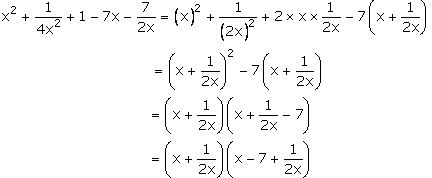= [x2 + 1/(2x)2 + 2 × x × 1/(2x)] – 7 [x + 1/(2x)]

= (x + 1/2x)2 – 7(x + 1/x)

Taking out (x + 1/2x) as common,

= (x + 1/2x) (x + 1/2x – 7)

2. 9a2 + 1/9a2 – 2 – 12a + 4/3a

Solution: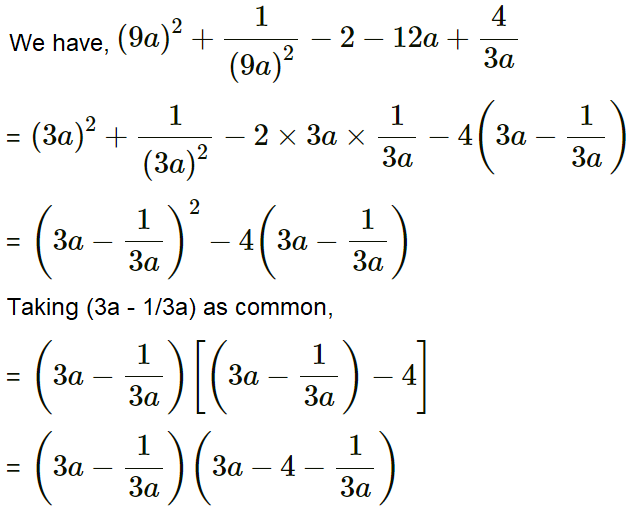3. x2 + (a2 + 1) x/a + 1

Solution: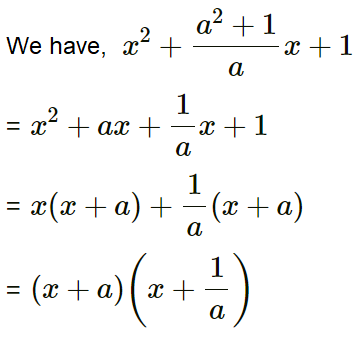4. x4 + y4 – 27x2y2

Solution:

We have, x4 + y4 – 27x2y2

= x4 + y4 – 2x2y2 – 25x2y2

= [(x2) + (y2) – 2x2y2] – 25x2y2

= (x2 – y2) – (5xy)2

= (x2 – y2 – 5xy) (x2 – y2 + 5xy) [As x2 – y2 = (x + y)(x – y)]

5. 4x4 + 9y4 + 11x2y2

Solution:

We have, 4x4 + 9y4 + 11x2y2

= 4x4 + 9y4 + 12x2y2 – x2y2

= (2x2)2 + (3y2)2 + 2(2x2)(3y2) – (xy)2

= (2x2 + 3y2)2 – (xy)2

= (2x2 + 3y2 – xy) (2x2 + 3y2 + xy) [As x2 – y2 = (x + y)(x – y)]

6. x2 + 1/x2 – 3

Solution:

We have, x2 + 1/x2 – 3

= x2 + 1/x2 – 2 – 1

= [x2 + 1/x2 – (2 × x × 1/x)] – 12

= (x – 1/x)2 – 12

= (x – 1/x – 1) (x – 1/x + 1) [As x2 – y2 = (x + y)(x – y)]

7. a – b – 4a2 + 4b2

Solution:

We have, a – b – 4a2 + 4b2

= (a – b) – 4(a2 – b2)

= (a – b) – 4(a – b)(a + b) [As x2 – y2 = (x + y)(x – y)]

Taking (a – b) common,

= (a – b) [1 – 4(a + b)]

= (a – b) [1 – 4a – 4b]

8. (2a – 3)2 – 2 (2a – 3) (a – 1) + (a – 1)2

Solution:

We have, (2a – 3)2 – 2(2a – 3)(a – 1) + (a – 1)2

Comparing with the identity, (a – b)2 = a2 – 2ab + b2

= [(2a – 3) – (a – 1)]2

= (2a – a – 3 + 1)2

= (a – 2)2

9. (a2 – 3a) (a2 – 3a + 7) + 10

Solution:

Let’s substitute (a2 – 3a) = x

Then the given expression becomes,

= x(x + 7) + 10

= x2 + 7x + 10

= x2 + 5x + 2x + 10 [By splitting the middle term]

= x(x + 5) + 2(x + 5)

= (x + 2) (x + 5)

Resubstituting the value of x,

= (a2 – 3a + 2) (a2 – 3a + 5)

= (a2 – 3a + 5) (a2 – 3a + 2)

= (a2 – 3a + 5) [a2 – 2a – a + 2] [By splitting the middle term]

= (a2 – 3a + 5) [a(a – 2) – 1(a – 5)]

= (a2 – 3a + 5) [(a – 1) (a – 2)]

= (a2 – 3a + 5) (a – 1) (a – 2)

10. (a2 – a) (4a2 – 4a – 5) – 6

Solution:

Let’s a2 – a = x

Then the expression becomes,

= x(4x – 5) – 6

= 4x2 – 5x – 6

= 4x2 – 8x + 3x – 6

= 4x(x – 2) + 3(x – 2)

= (4x + 3) (x – 2)

Resubstituting the value of x,

= (4a2 – 4a + 3) (a2 – a – 2)

= (4a2 – 4a + 3) (a2 – 2a + a – 2)

= (4a2 – 4a + 3) [a(a – 2) + 1(a – 2)]

= (4a2 – 4a + 3) [(a + 1) (a – 2)]

= (4a2 – 4a + 3) (a + 1) (a – 2)

11. x4 + y4 – 3x2y2

Solution:

We have, x4 + y4 – 3x2y2

= (x4 + y4 – 2x2y2) – x2y2

= (x2 – y2) – (xy)2 [As x2 – y2 = (x + y)(x – y)]

= (x2 – y2 – xy) (x2 – y2 + xy)

12. 5a2 – b2 – 4ab + 7a – 7b

Solution:

We have, 5a2 – b2 – 4ab + 7a – 7b

= 4a2 + a2 – b2 – 4ab + 7a – 7b

= a2 – b2 + 4a2 – 4ab + 7a – 7b

= (a2 – b2) + 4a(a – b) + 7(a – b)

= (a + b)(a – b) + 4a(a – b) + 7(a – b) [As x2 – y2 = (x + y)(x – y)]

= (a – b) [(a + b) + 4a + 7]

= (a – b) (5a + b + 7)

13. 12(3x – 2y)2 – 3x + 2y – 1

Solution:

We have, 12(3x – 2y)2 – 3x + 2y – 1

= 12(3x – 2y)2 – (3x – 2y) – 1

Let’s substitute (3x – 2y) = a

Then, the expression becomes

= 12a2 – a – 1

= 12a2 – 4a + 3a – 1

= 4a (3a – 1) + 1(3a – 1)

= (4a + 1) (3a – 1)

Now, resubstituting the value of ‘a’ in the above

= [4(3x – 2y) + 1] [3(3x – 2y) – 1]

= (12x – 8y + 1) (9x – 6y – 1)

14. 4(2x – 3y)2 – 8x+12y – 3

Solution:

We have, 4(2x – 3y)2 – 8x+12y – 3

= 4(2x – 3y)2 – 4(2x + 3y) – 3

Let’s substitute (2x – 3y) = a

= 4(a2) – 4a – 3

= 4a2 – 6a + 2a – 3 [By splitting the middle term]

= 2a(2a – 3) + 1(2a – 3)

= (2a – 3) (2a + 1)

Now, resubstituting the value of ‘a’ in the above

= [2(2x – 3y) – 3] [2(2x – 3y) + 1]

= (4x – 6y – 3) (4x – 6y + 1)

15. 3 – 5x + 5y – 12(x – y)2

Solution:

We have, 3 – 5x + 5y – 12(x – y)2

= 3 – 5(x – y) – 12(x – y)2

Let’s substitute (x – y) = a

= 3 – 5a – 12a2

= 3 – 9a + 4a – 12a2 [By splitting the middle term]

= 3(1 – 3a) + 4a(1 – 3a)

= (1 – 3a) (4a + 3)

Now, resubstituting the value of ‘a’ in the above

= [1 – 3(x – y)] [4(x – y) + 3]

= (1 – 3x + 3y) (4x – 4y + 3)

16. 9x 2 + 3x – 8y – 64y2

Solution:

We have, 9x 2 + 3x – 8y – 64y2

On rearranging,

= 9x 2 – 64y2 + 3x – 8y

= [(3x)2 – (8y)2] + (3x – 8y)

= (3x – 8y) (3x + 8y) + (3x – 8y) [As x2 – y2 = (x + y)(x – y)]

Taking (3x – 8y) as common,

= (3x – 8y) (3x + 8y + 1)

17. 2√3x2 + x – 5√3

Solution:

We have, 2√3x2 + x – 5√3

By splitting the middle term,

= 2√3x2 + 6x – 5x – 5√3

= 2√3x(x + √3) – 5(x + √3)

= (2√3x – 5) (x + √3)

18. ¼ (a + b)2 – 9/16 (2a – b)2

Solution: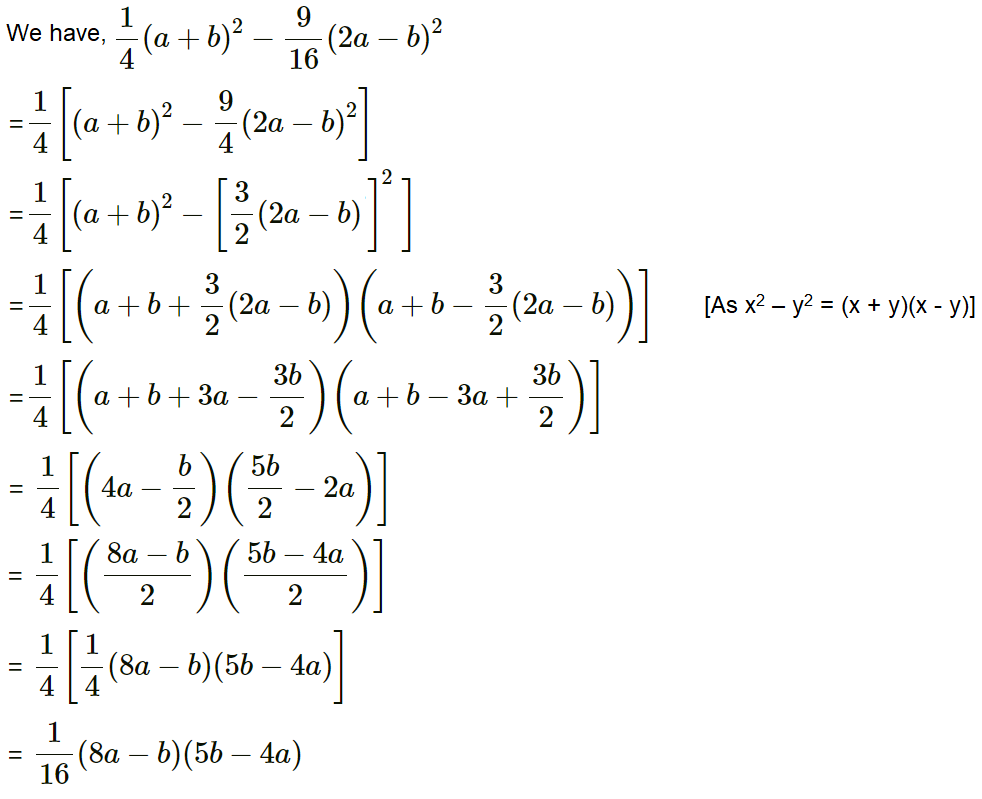19. 2(ab + cd) – a2 – b2 + c2 + d2

Solution:

We have, 2(ab + cd) – a2 – b2 + c2 + d2

= 2ab + 2cd – a2 – b2 + c2 + d2

On rearranging and grouping, we get

= (c2 + d2 + 2cd) – (a2 + b2 – 2ab)

= (c + d)2 – (a – b)2

= [c + d – (a – b)] [c + d + (a – b)] [As x2 – y2 = (x + y)(x – y)]

= (c + d – a + b) (c + d + a – b)

20. Find the value of:

(i) 9872 – 132

(ii) (67.8)2 – (32.2)2

(iii) [(6.7)2 – (3.3)2]/ (6.7 – 3.3)

(iv) [(18.5)2 – (6.5)2]/ (18.5 – 6.5)

Solution:

(i) We have, 9872 – 132

= (987 + 13) (987 – 13)

= 1000 x 974

= 974000

(ii) We have, (67.8)2 – (32.2)2

= (67.8 + 32.2) (67.8 – 32.2)

= 100 x 35.6

= 3560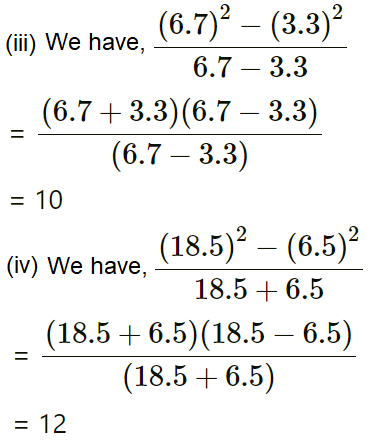## Selina Solutions for Class 9 Maths Chapter 5- Factorisation

Chapter 5, Factorisation, contains 5 exercises and the Solutions given here contains the answers for all the questions present in these exercises. Let us have a look at some of the topics that are discussed in this chapter.

5.1 Introduction

5.2 Methods of factorisation

Type 1: Taking out the common factors

Type 2: Grouping

Type 3: Trinomial of the form ax2+bx+c (by splitting method)

Type 4: Difference of two squares

Type 5: The sum or difference of two cubes

## Selina Solutions for Class 9 Maths Chapter 5- Factorisation

In Chapter 5 of Class 9, the students are taught factorisation. When a polynomial is expressed as the product of two or more expressions, each of these expressions is called a factor of the polynomial. The chapter helps the students in learning the process of writing an expression in the form of terms or brackets multiplied together, the process of factorisation. Study Chapter 5 of Selina textbook to understand more about Factorisation. Learn the Selina Solutions for Class 9 effectively to come out with excellent marks in the examinations.